# MSBSHSE Solutions For Class 9 Maths Part 2 Chapter 3 Triangles

MSBSHSE Solutions For Class 9 Maths Part 2 Chapter 3 Triangles consists of accurate solutions, which helps the students to quickly complete their homework and prepare well for the exams. BYJU’S provides clear and step by step solutions designed by our dedicated specialists. Students can easily master the concepts of Triangles. We come across the Theorem of remote interior angles, Congruence of triangles and Theorem of isosceles triangle in this chapter. MSBSHSE Solutions For Class 9 Maths Part 2 Chapter 3 Triangles helps students to easily ace the concepts of Triangles. Solutions are available in PDF format on our website.

## Download the PDF of Maharashtra Solutions For Class 9 Maths Part 2 Chapter 3 Triangles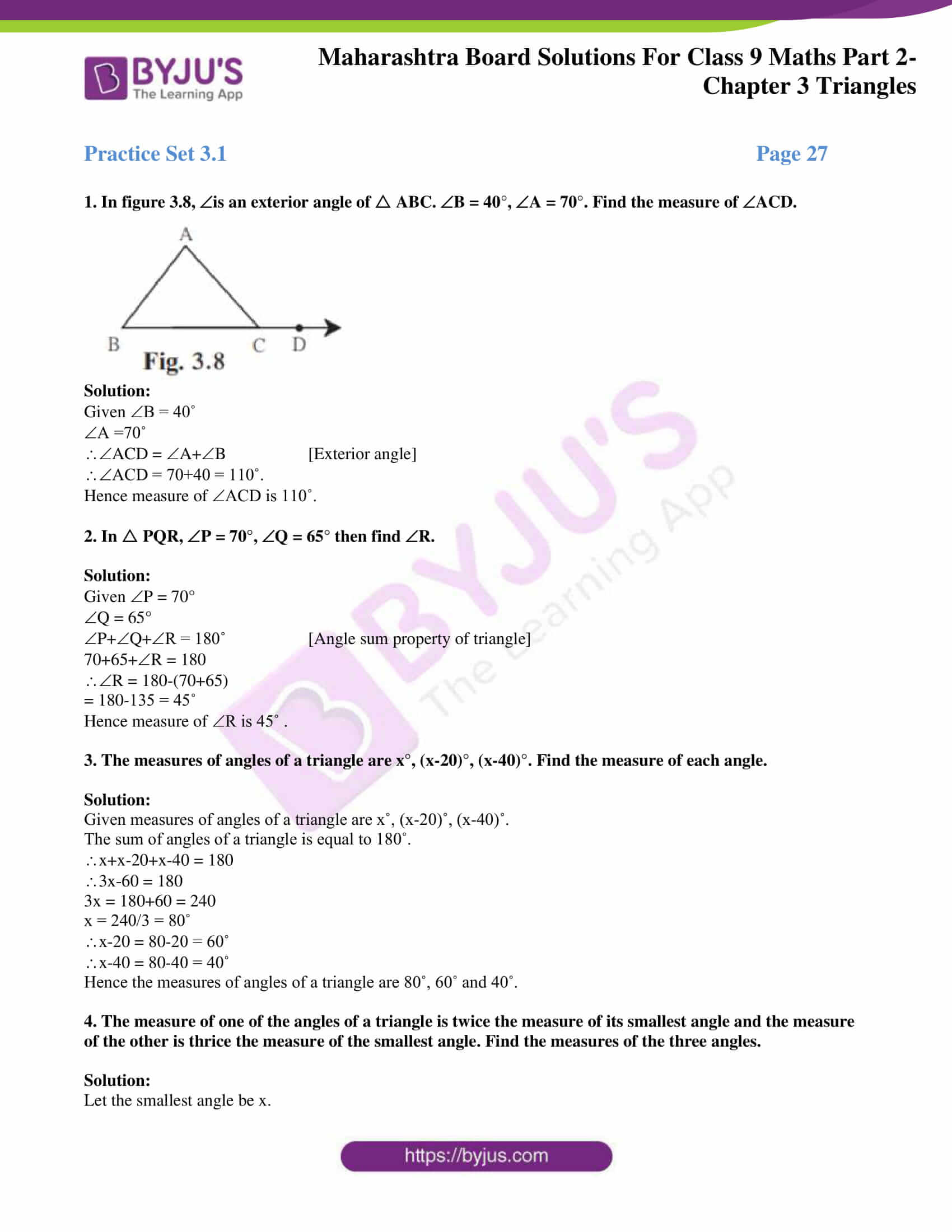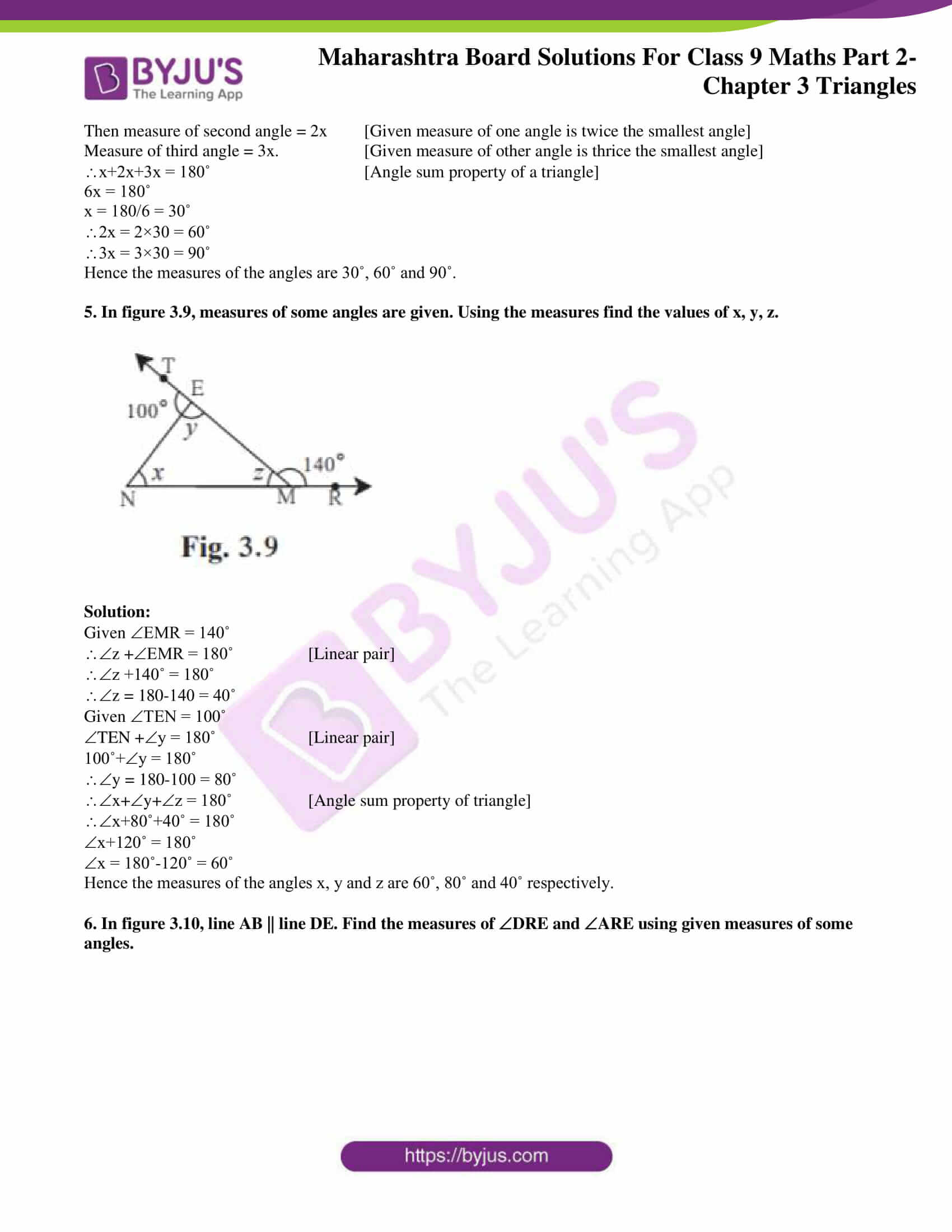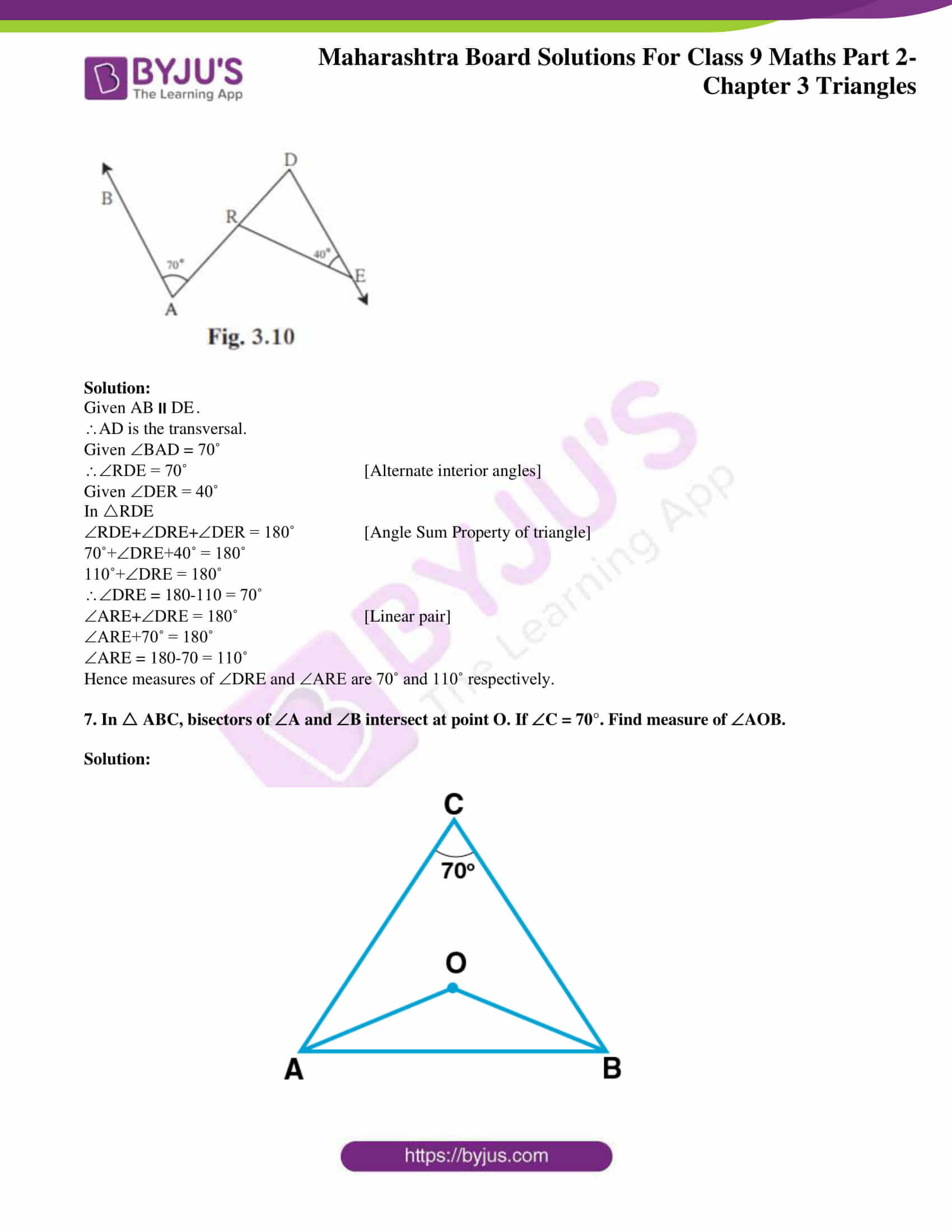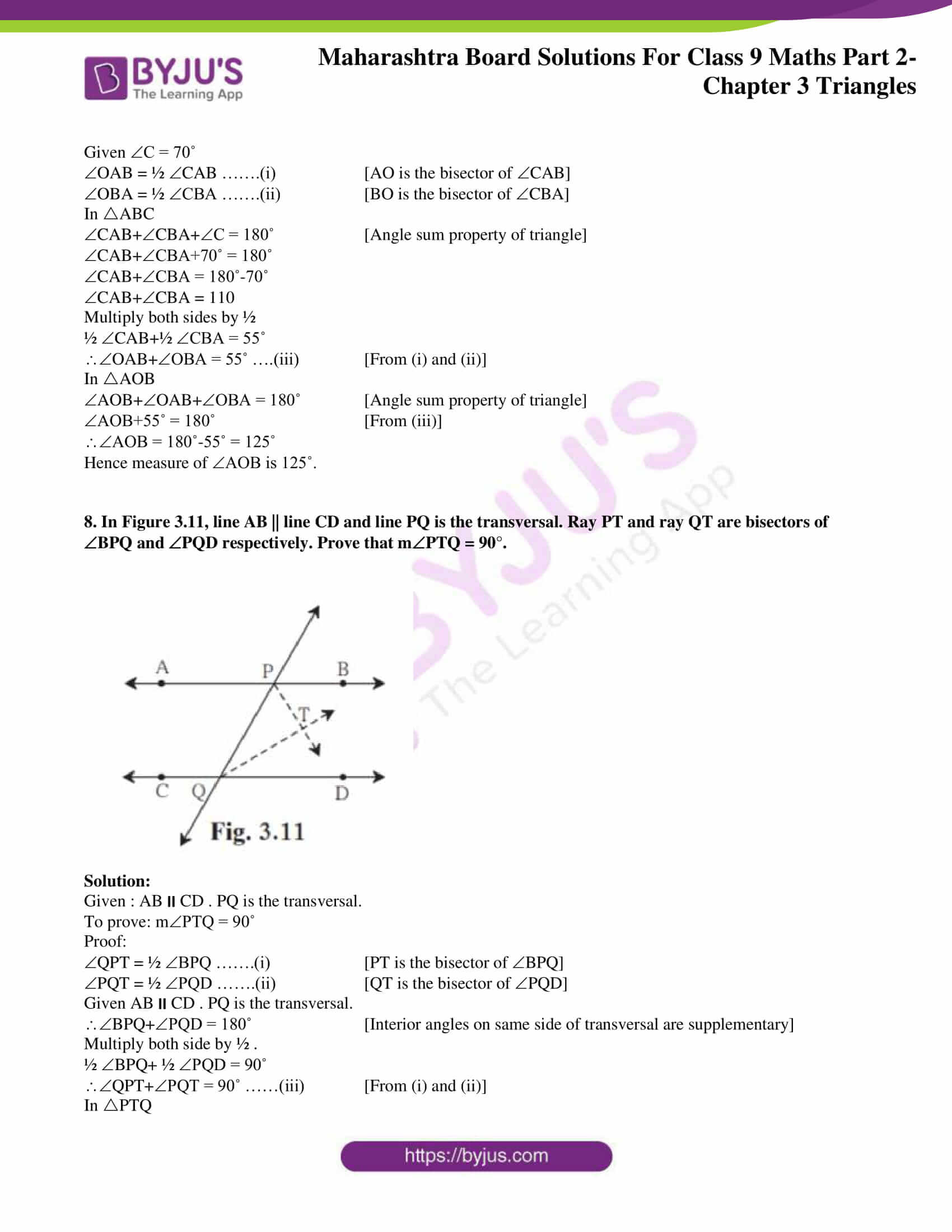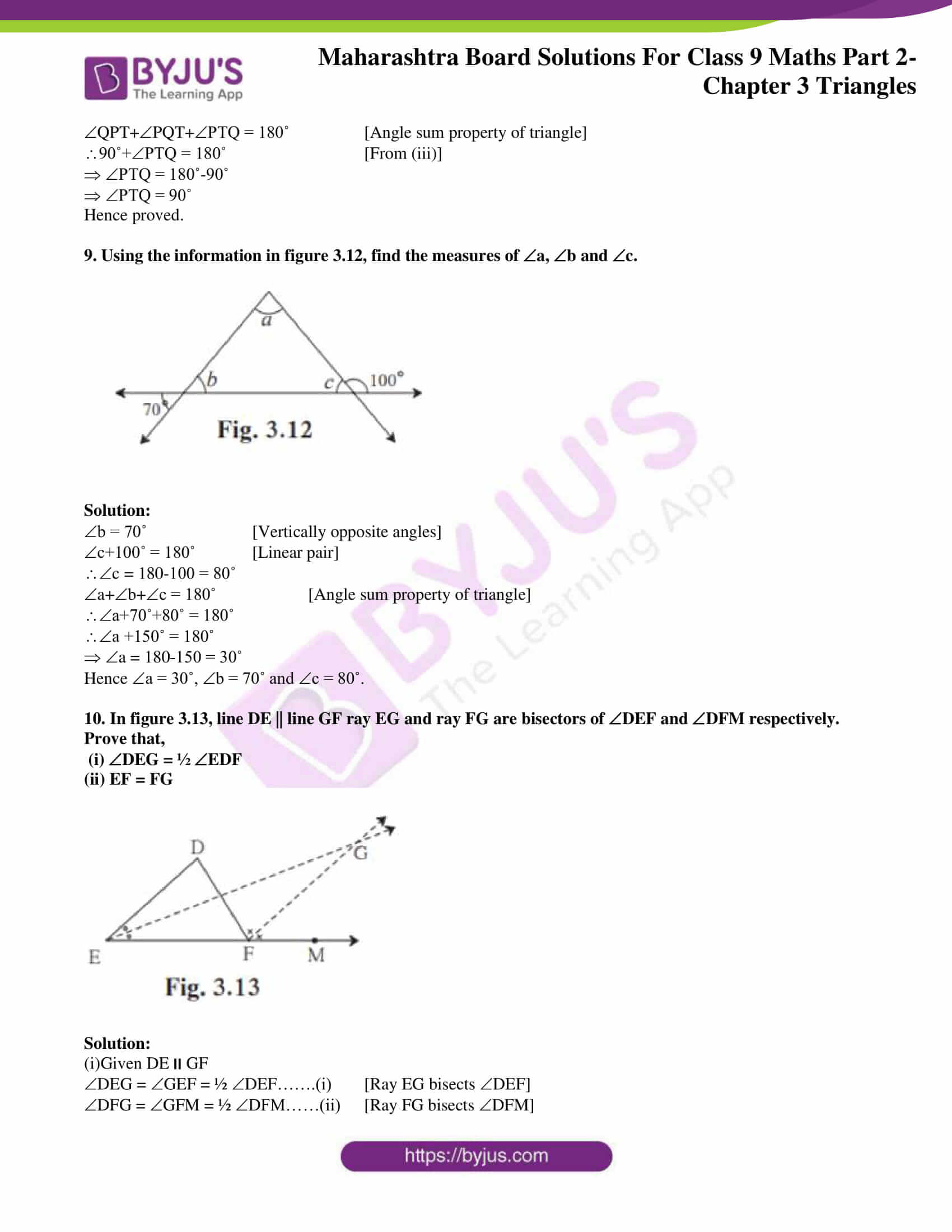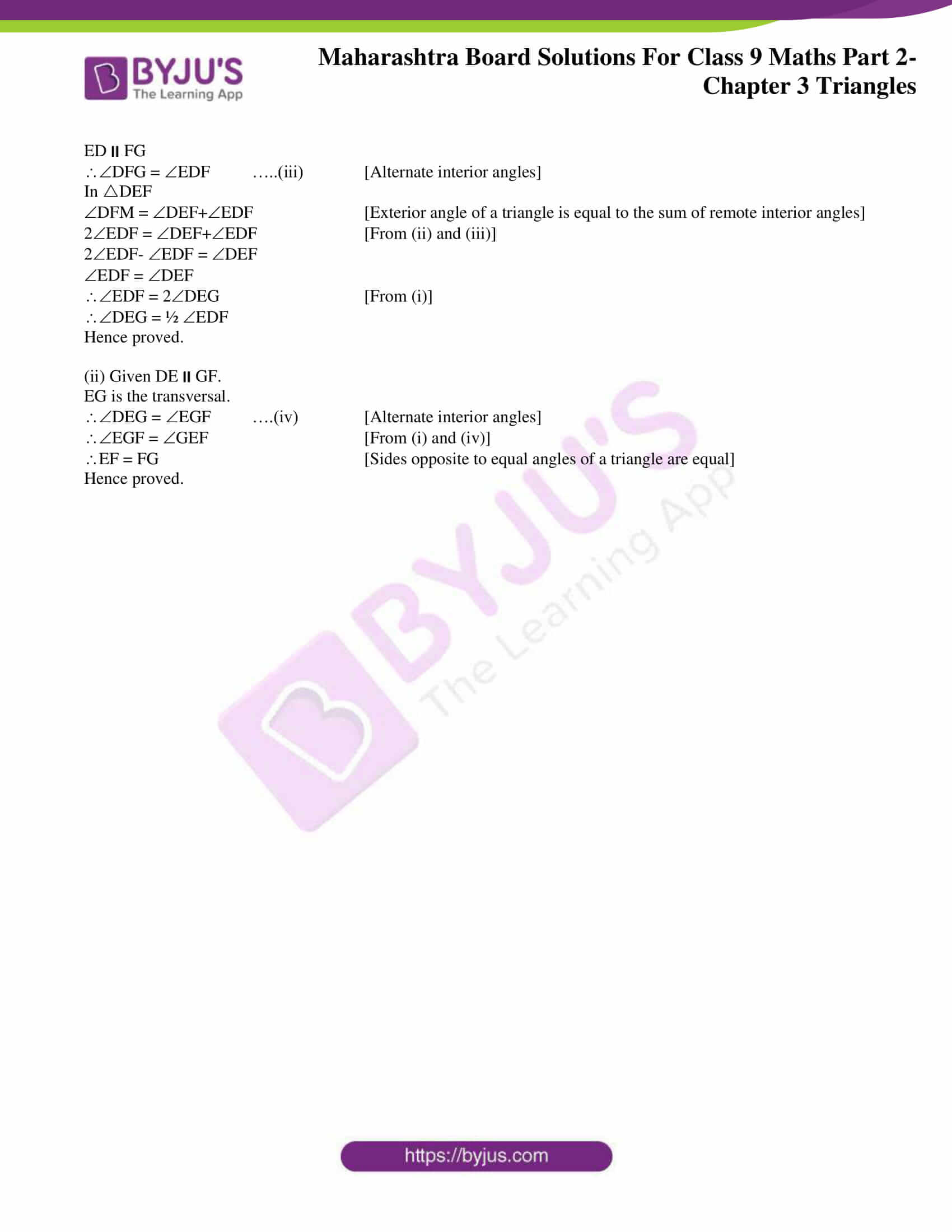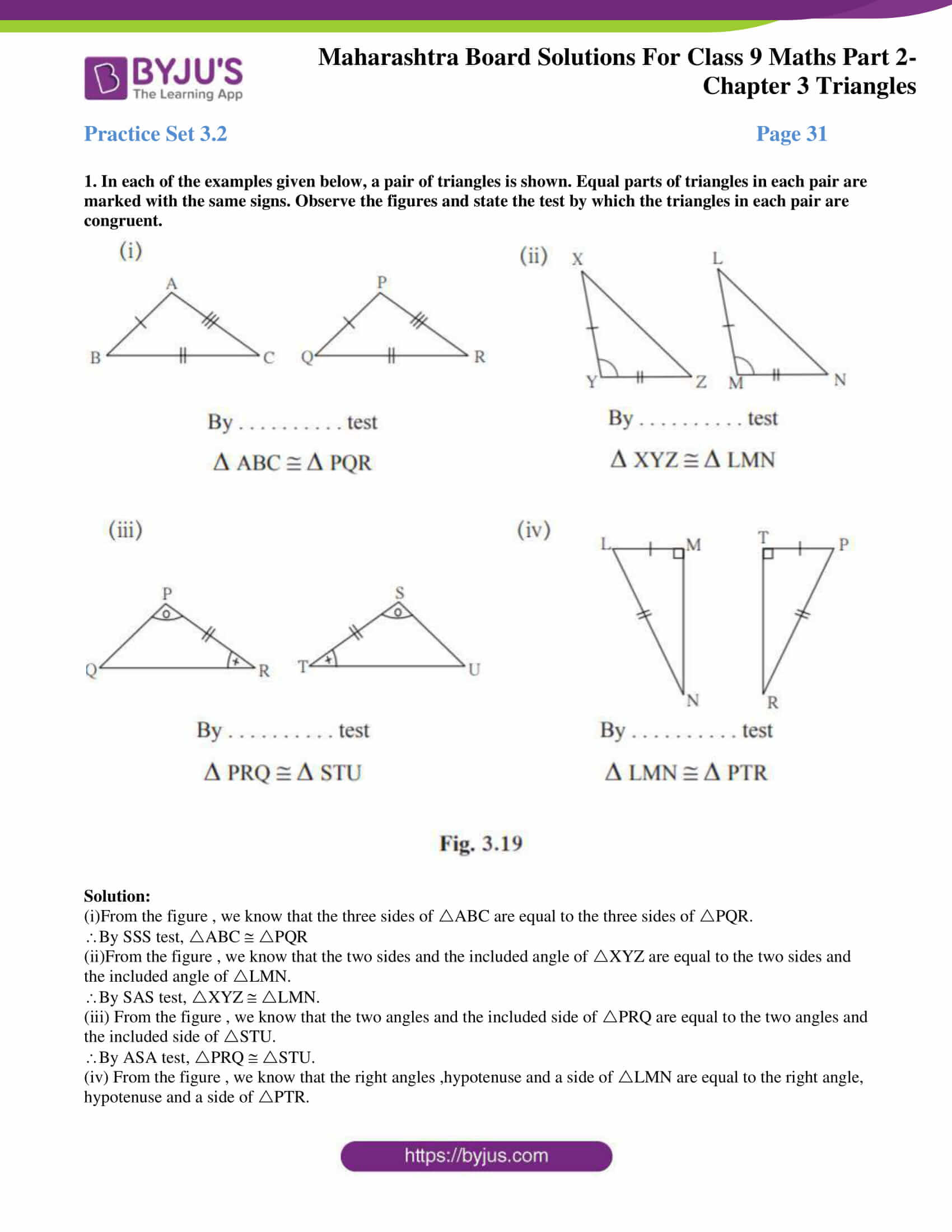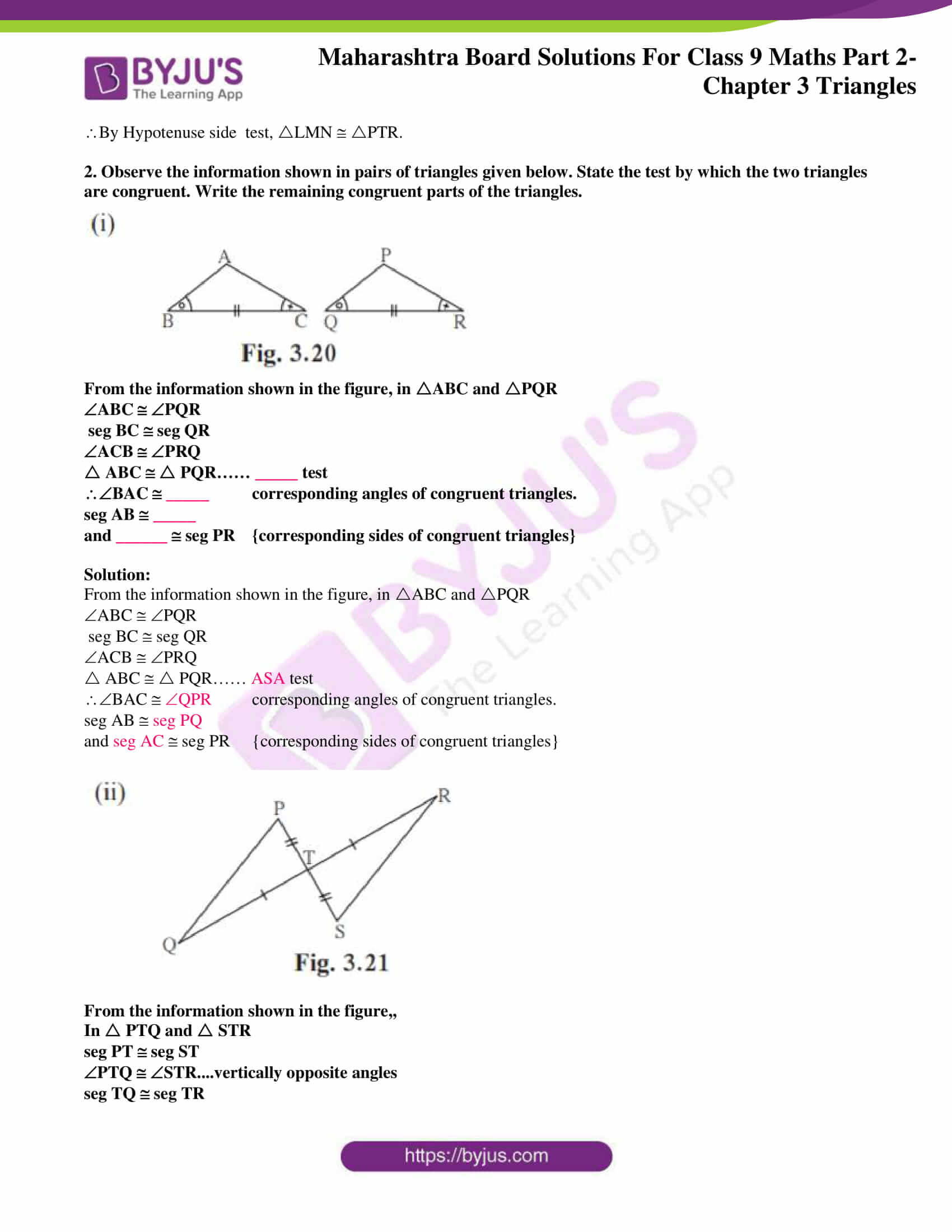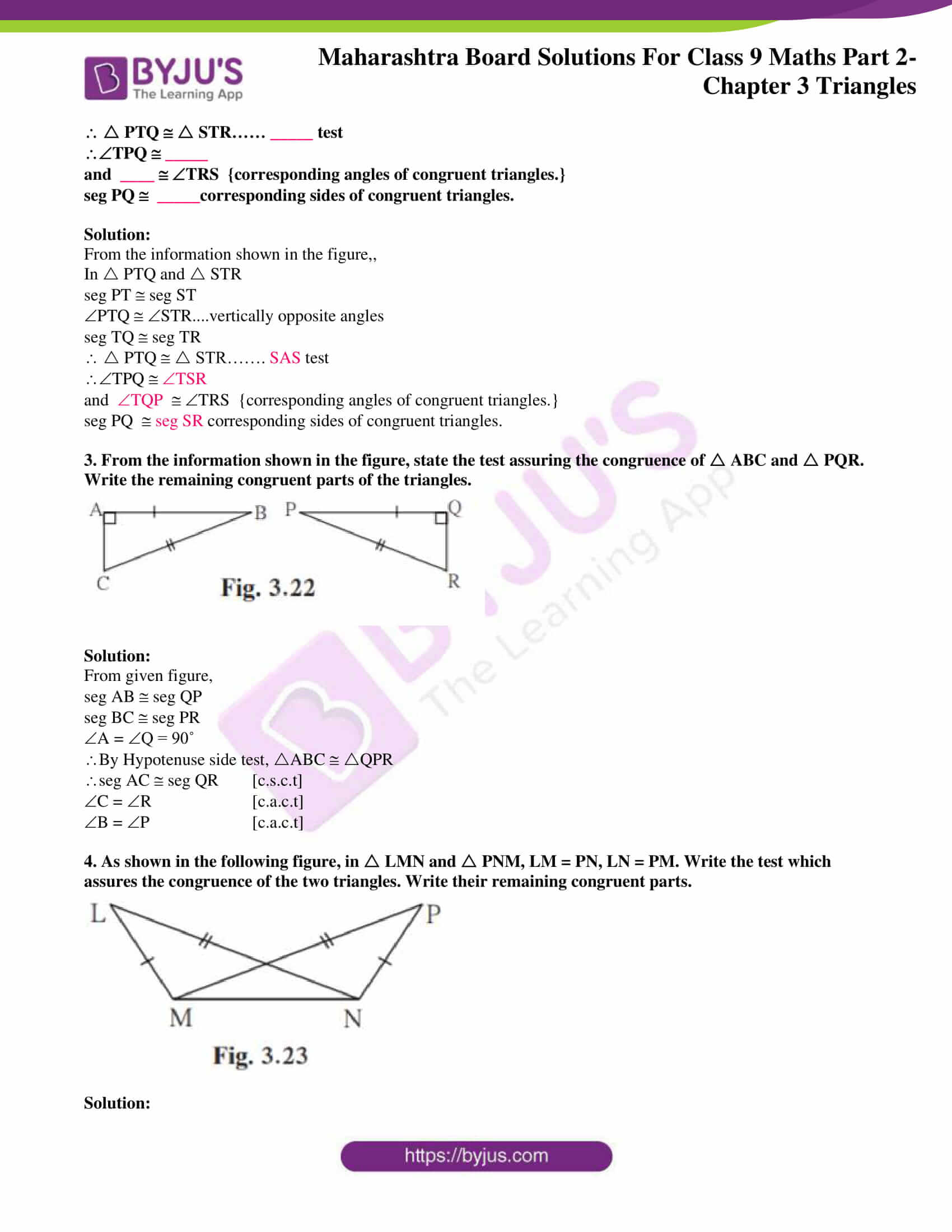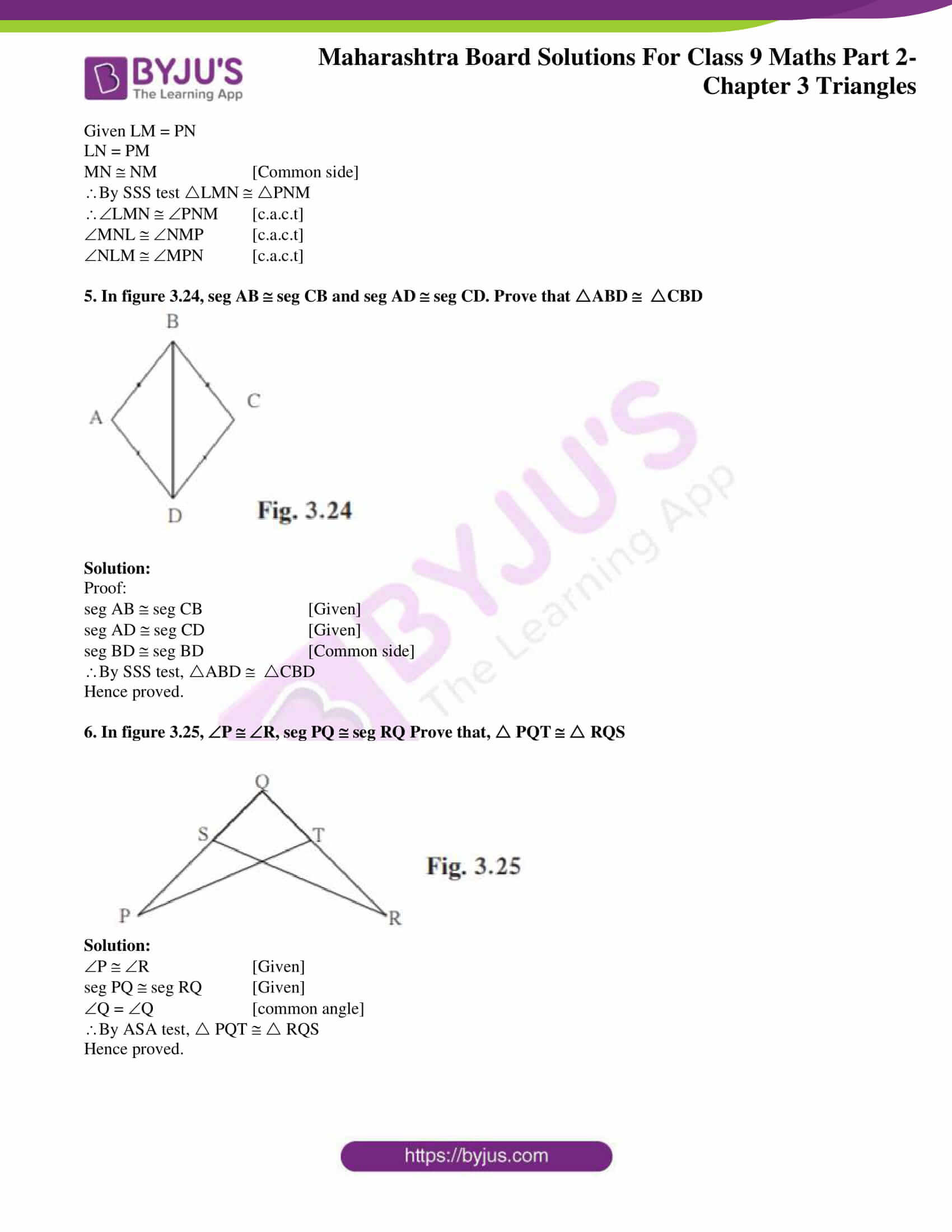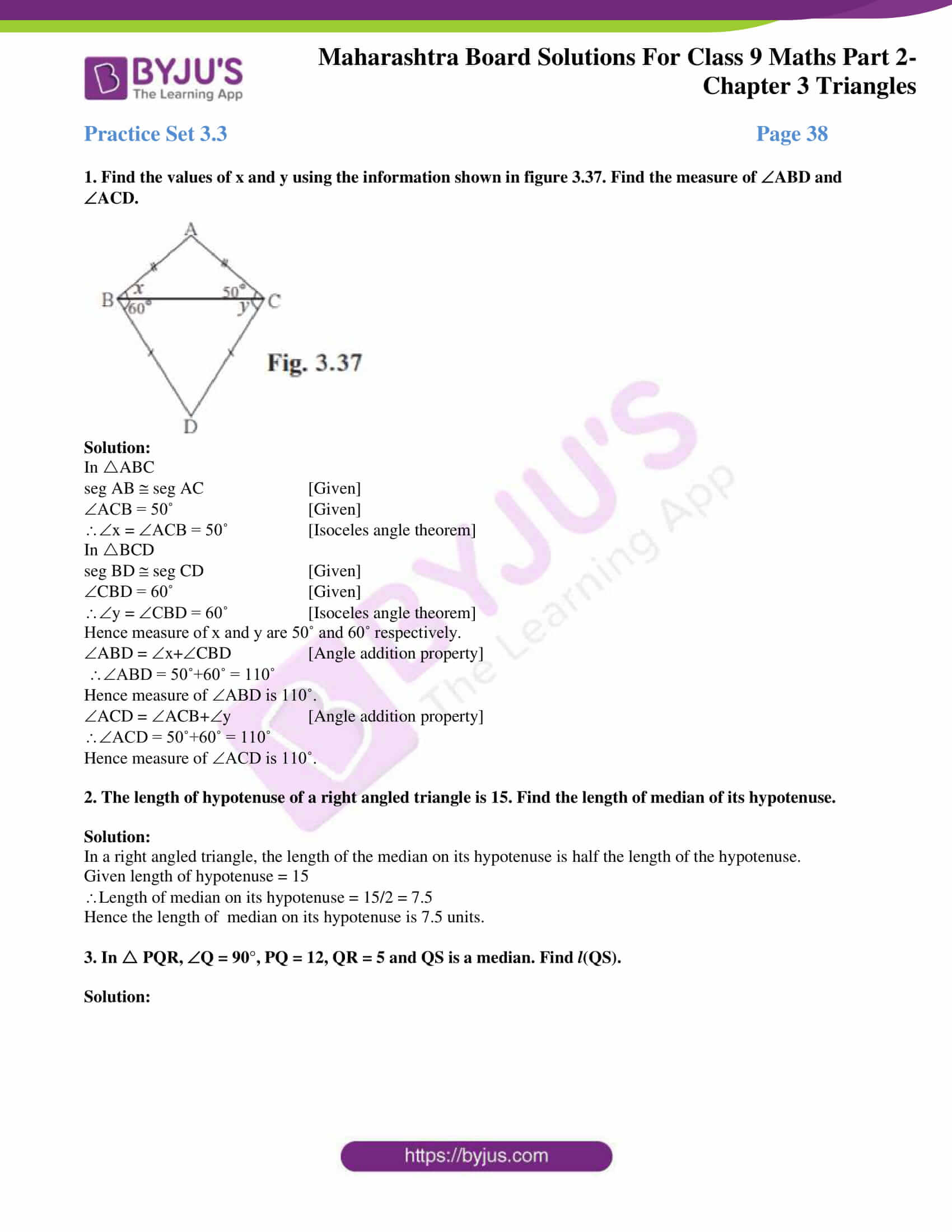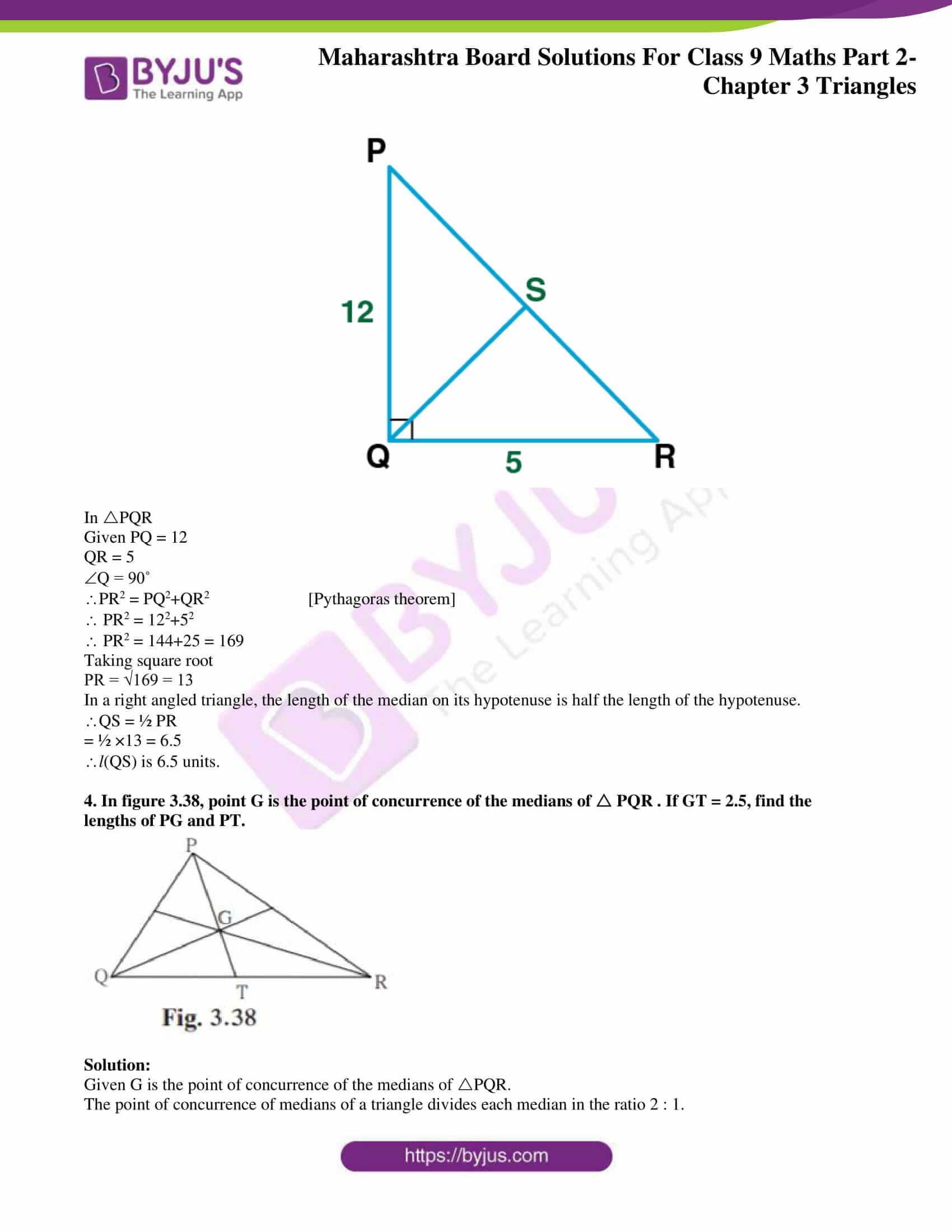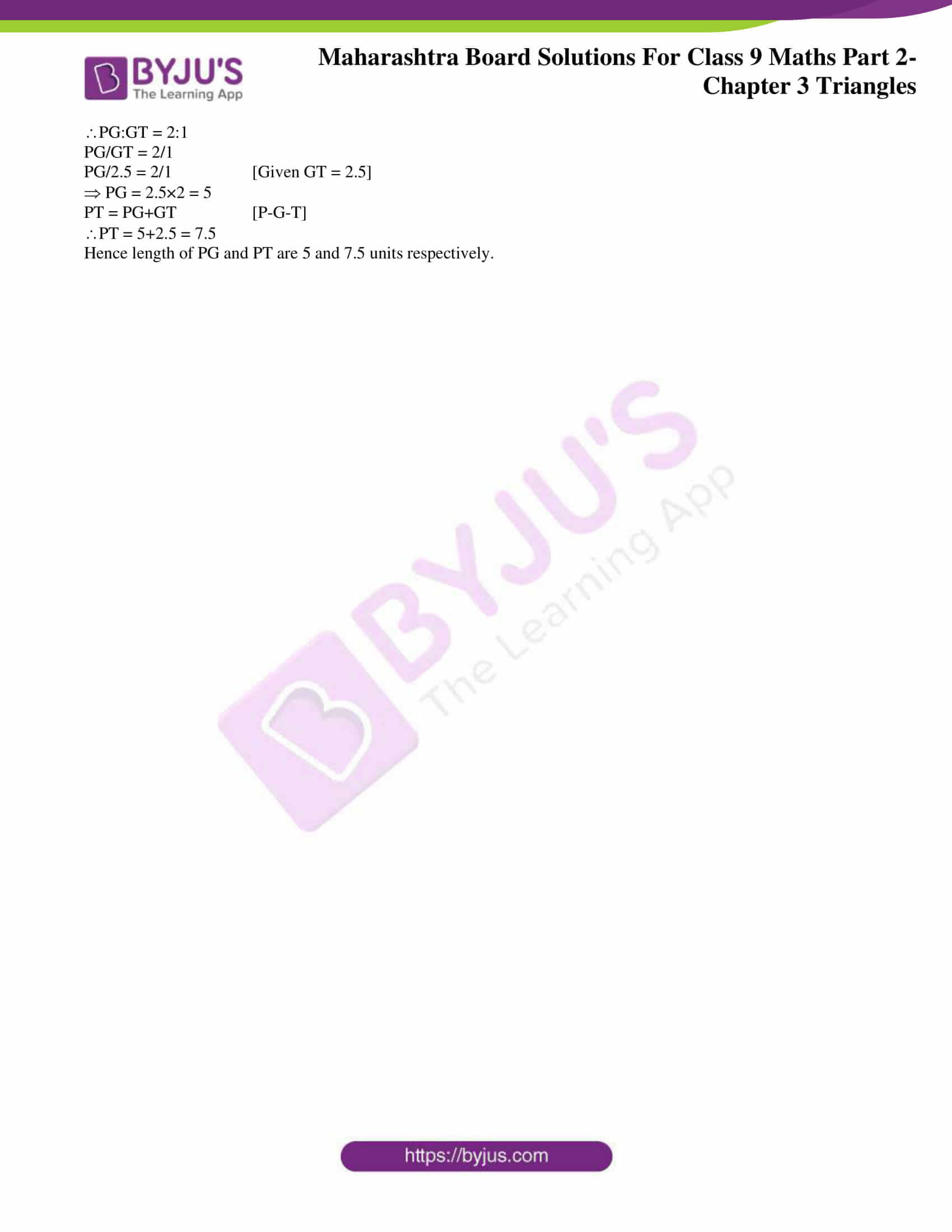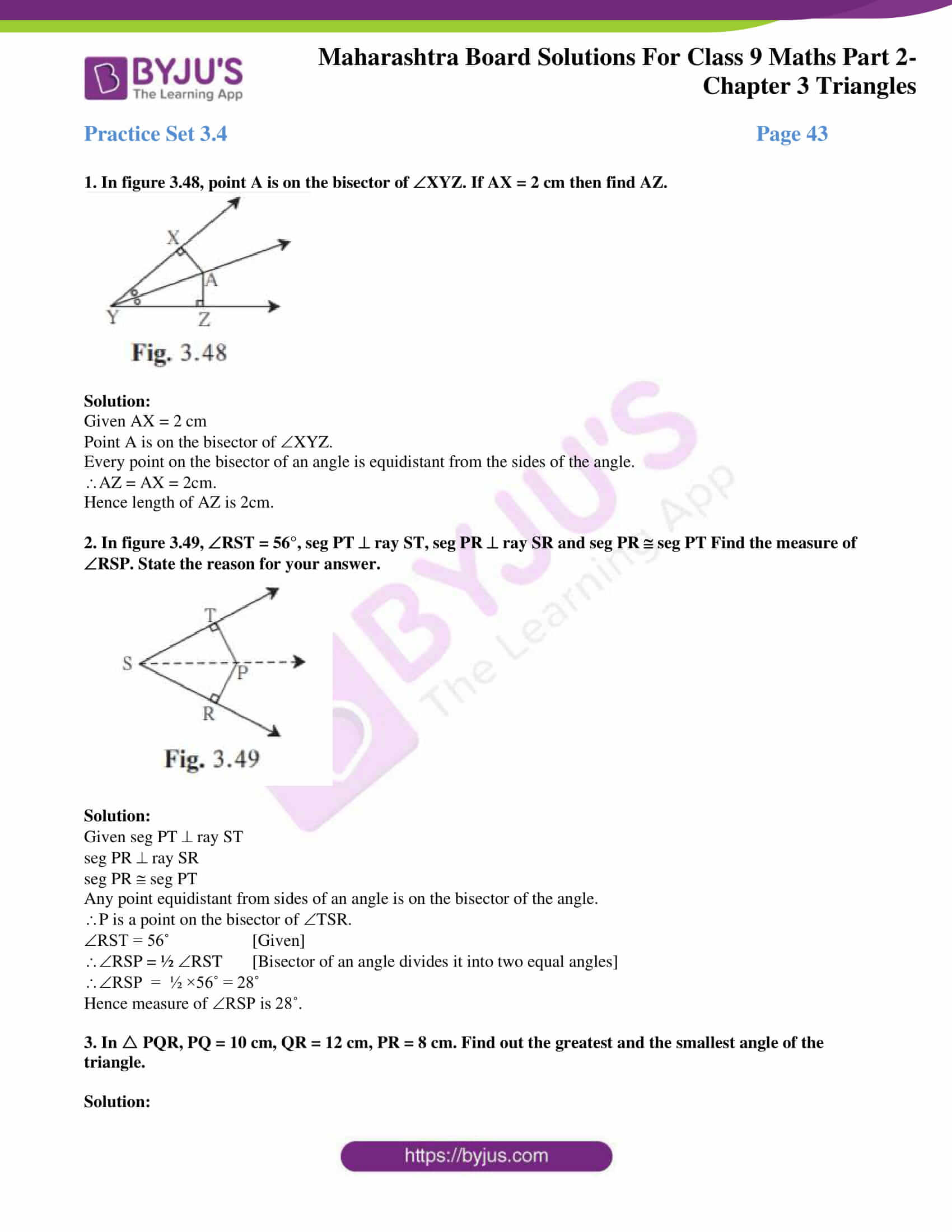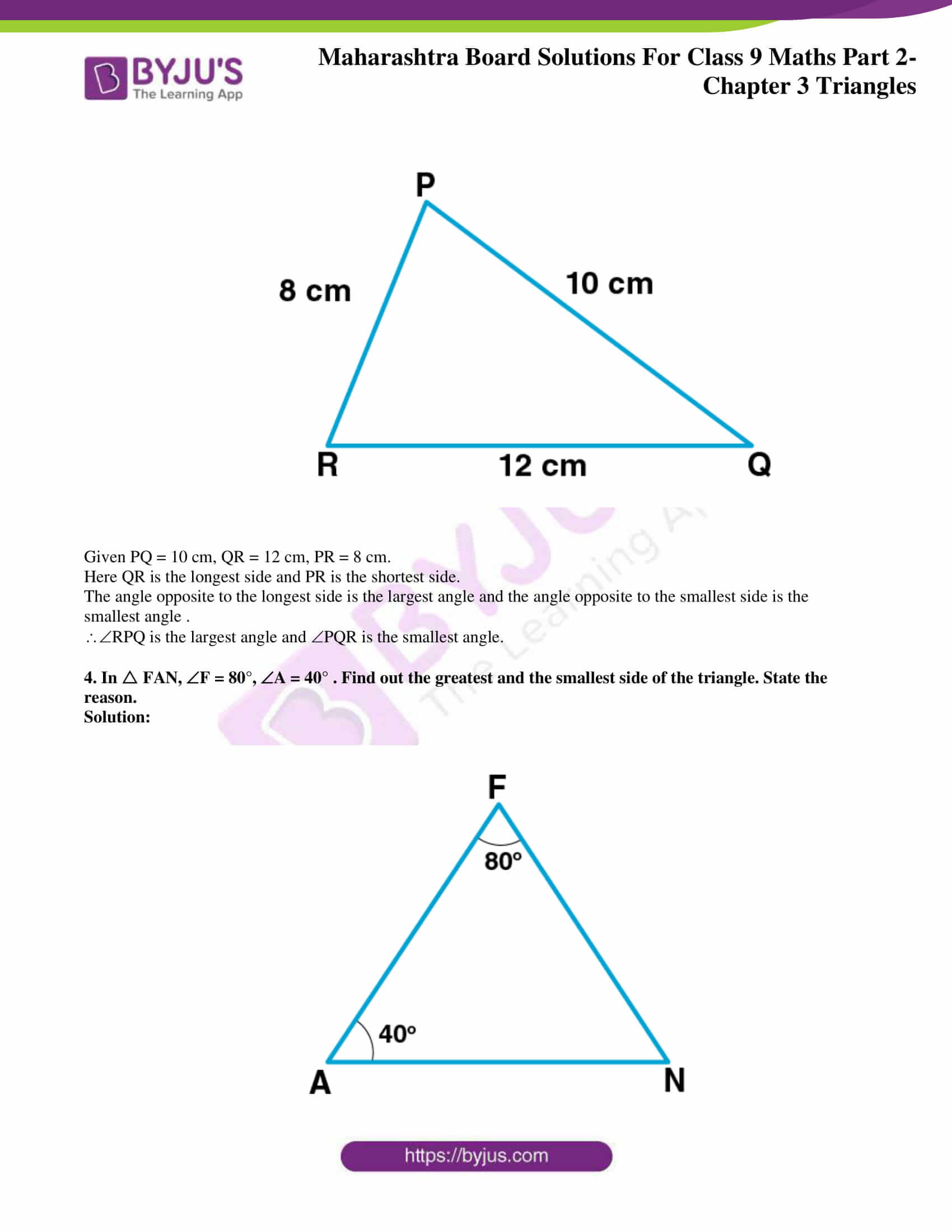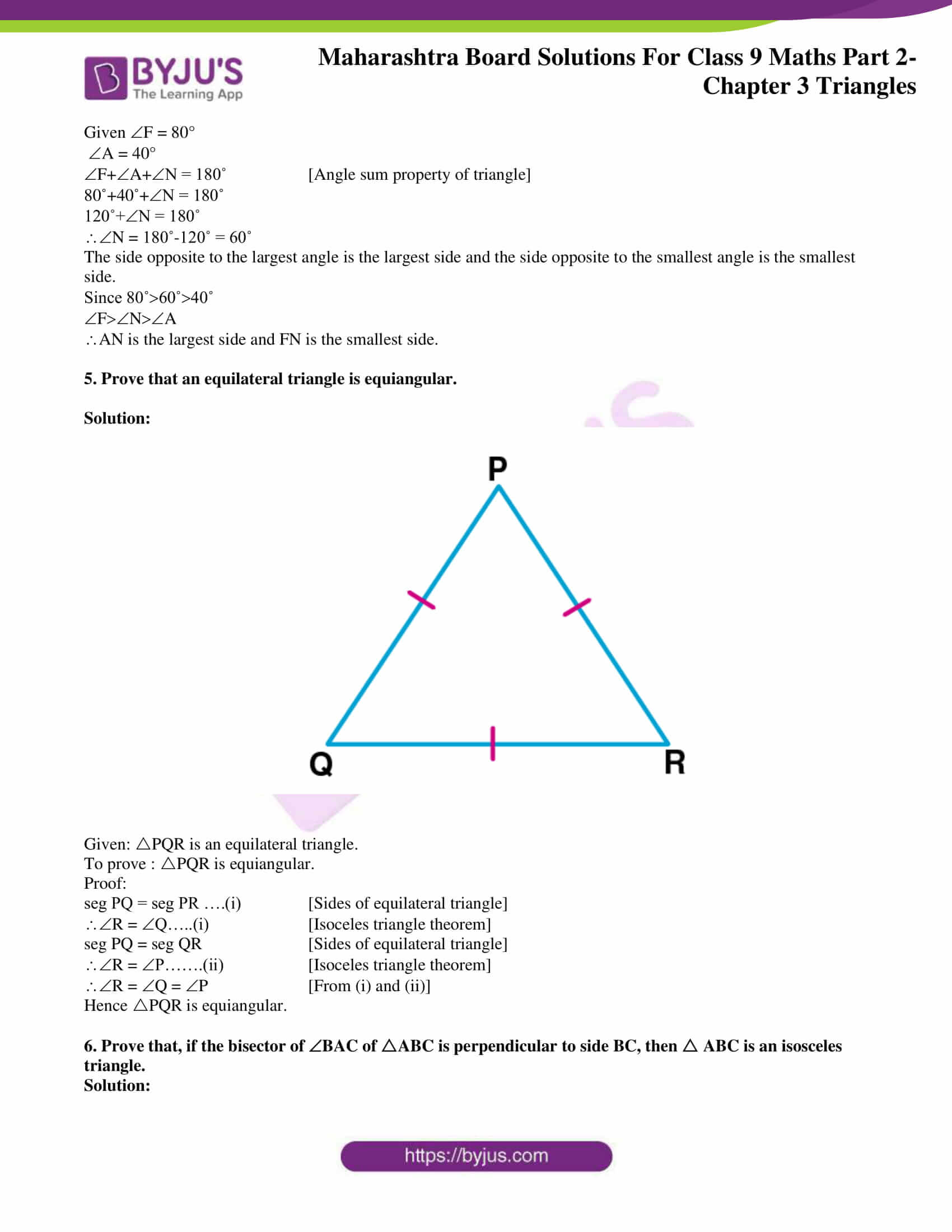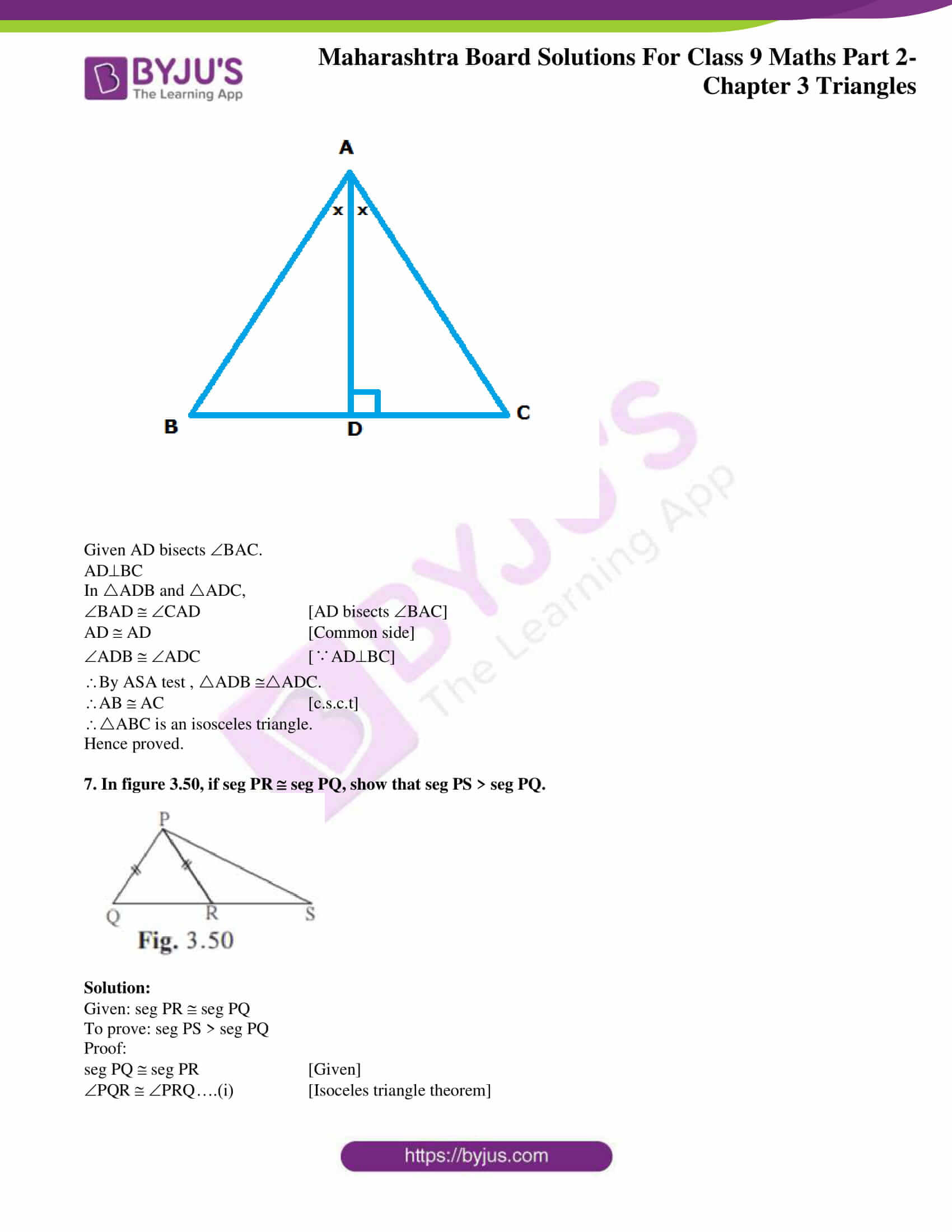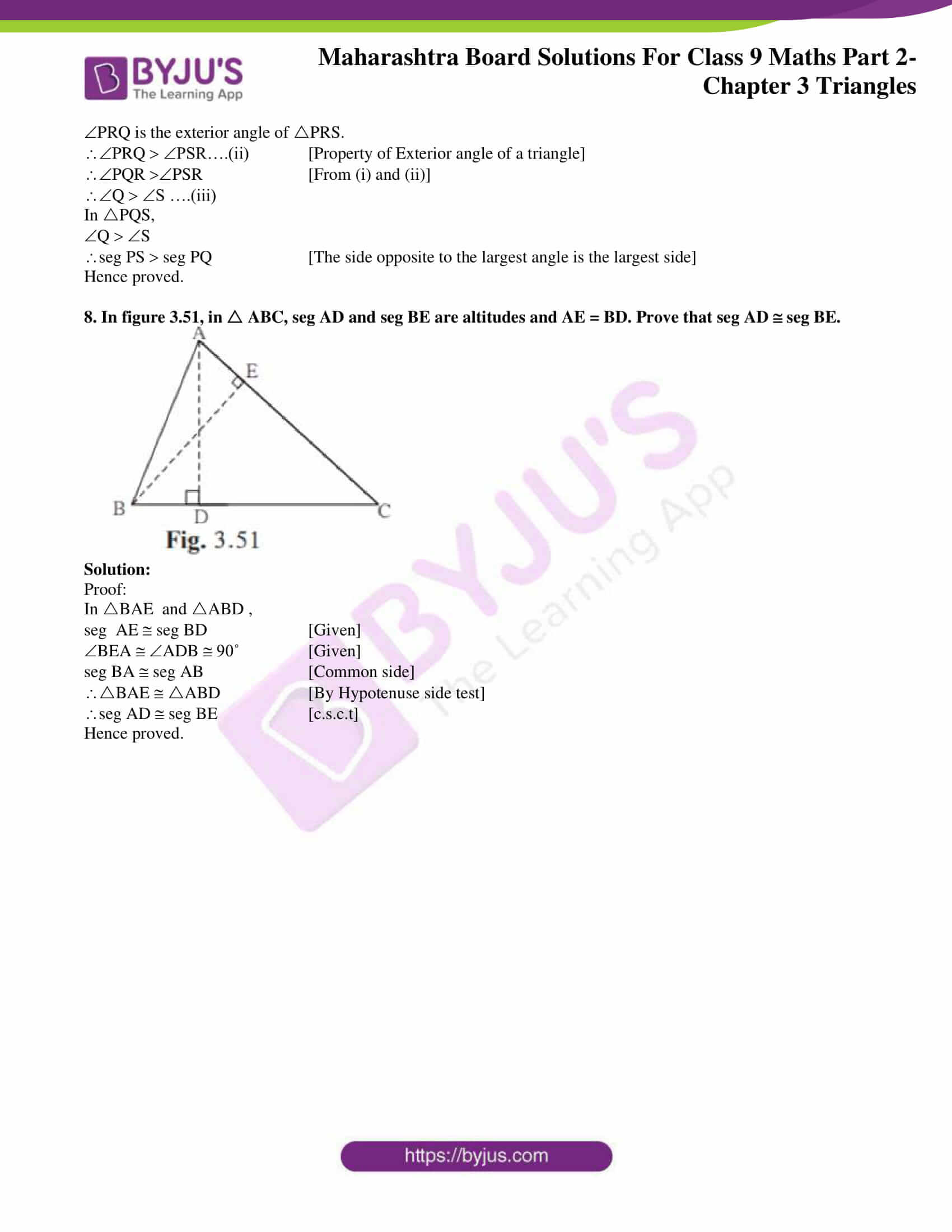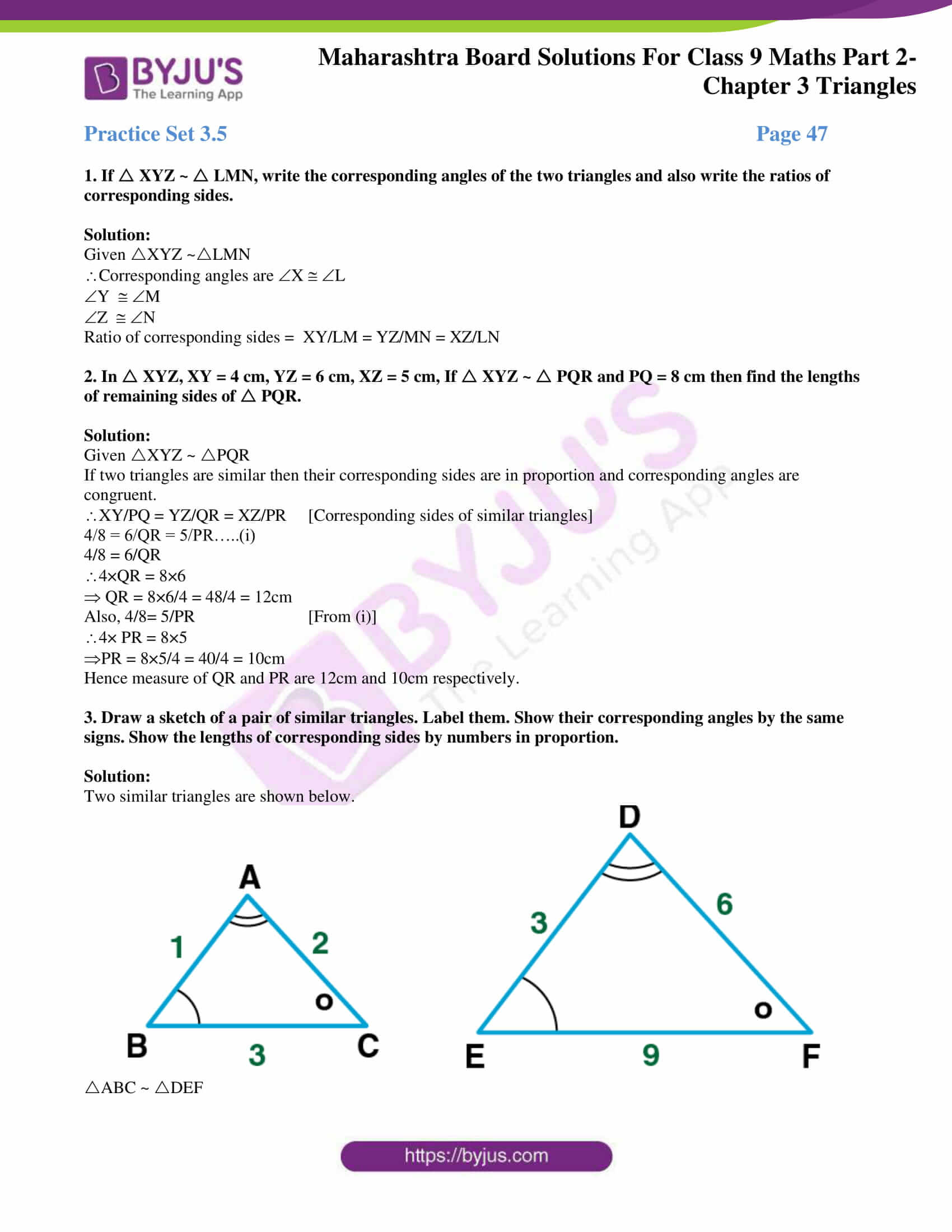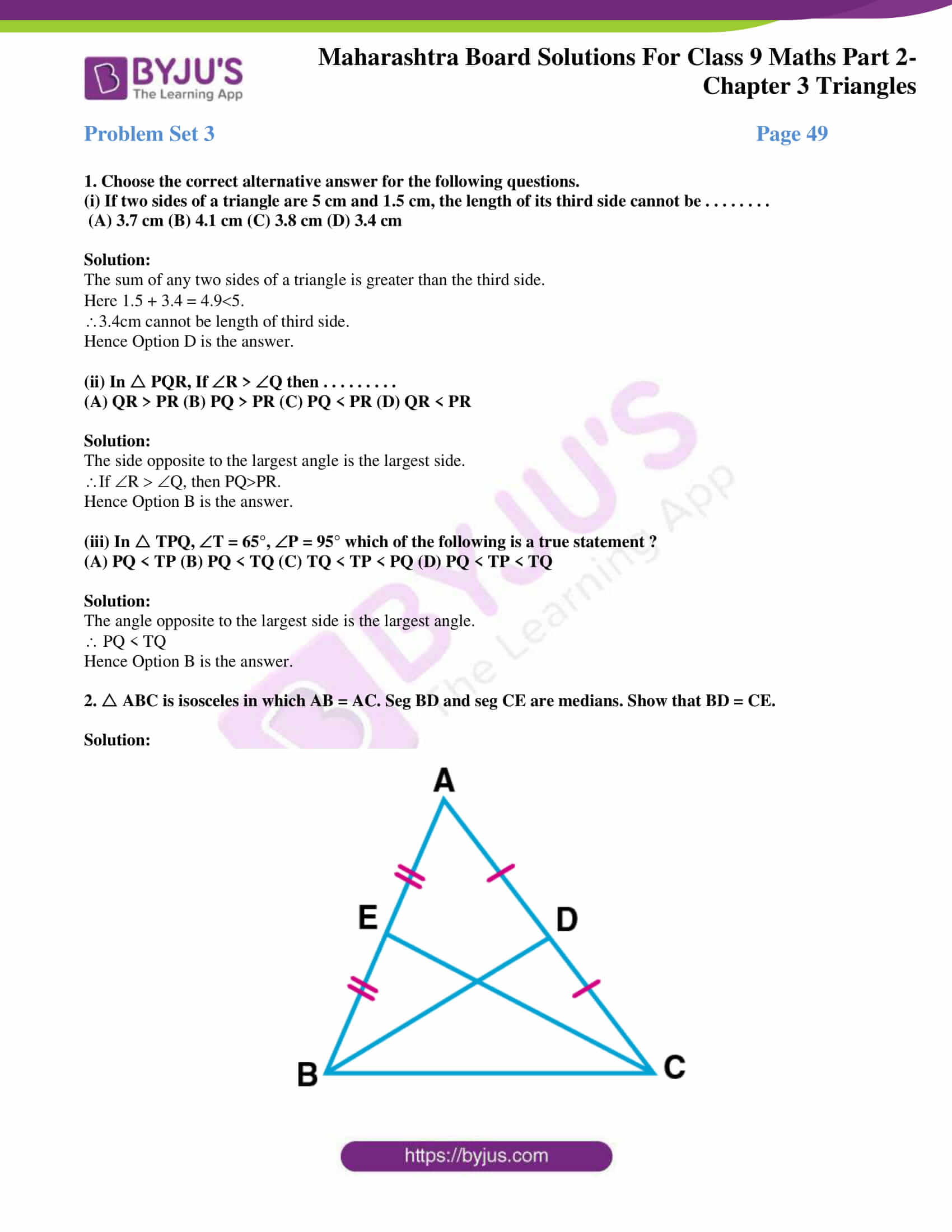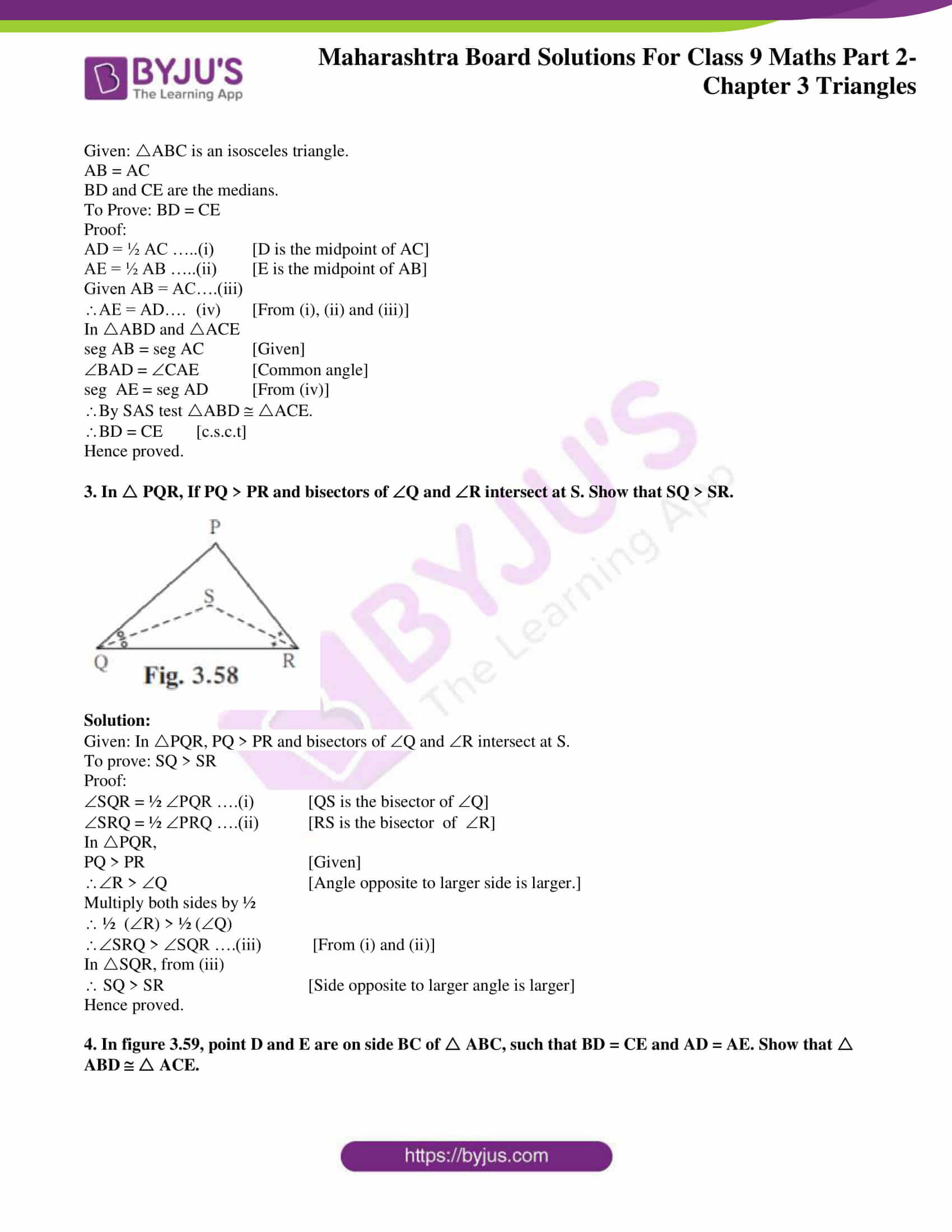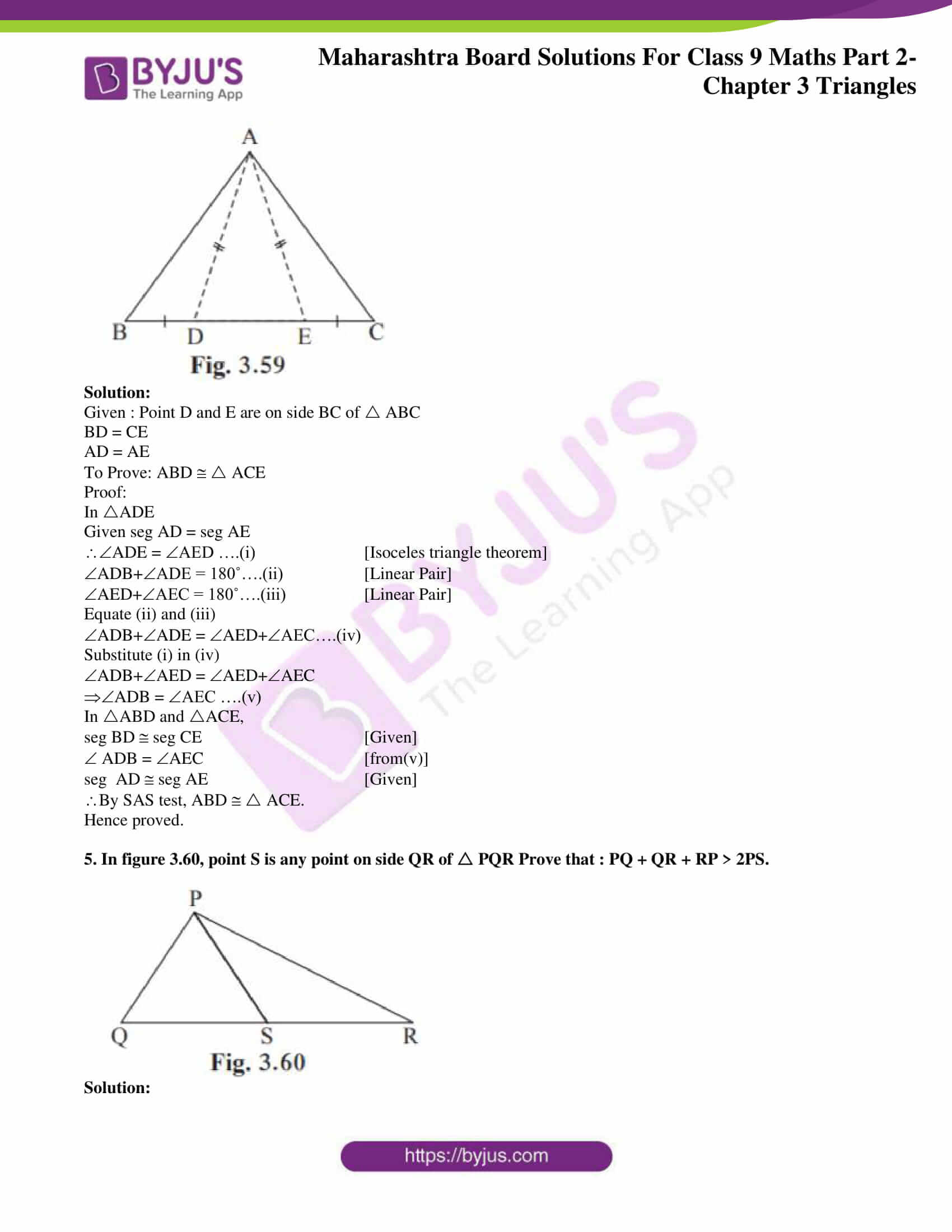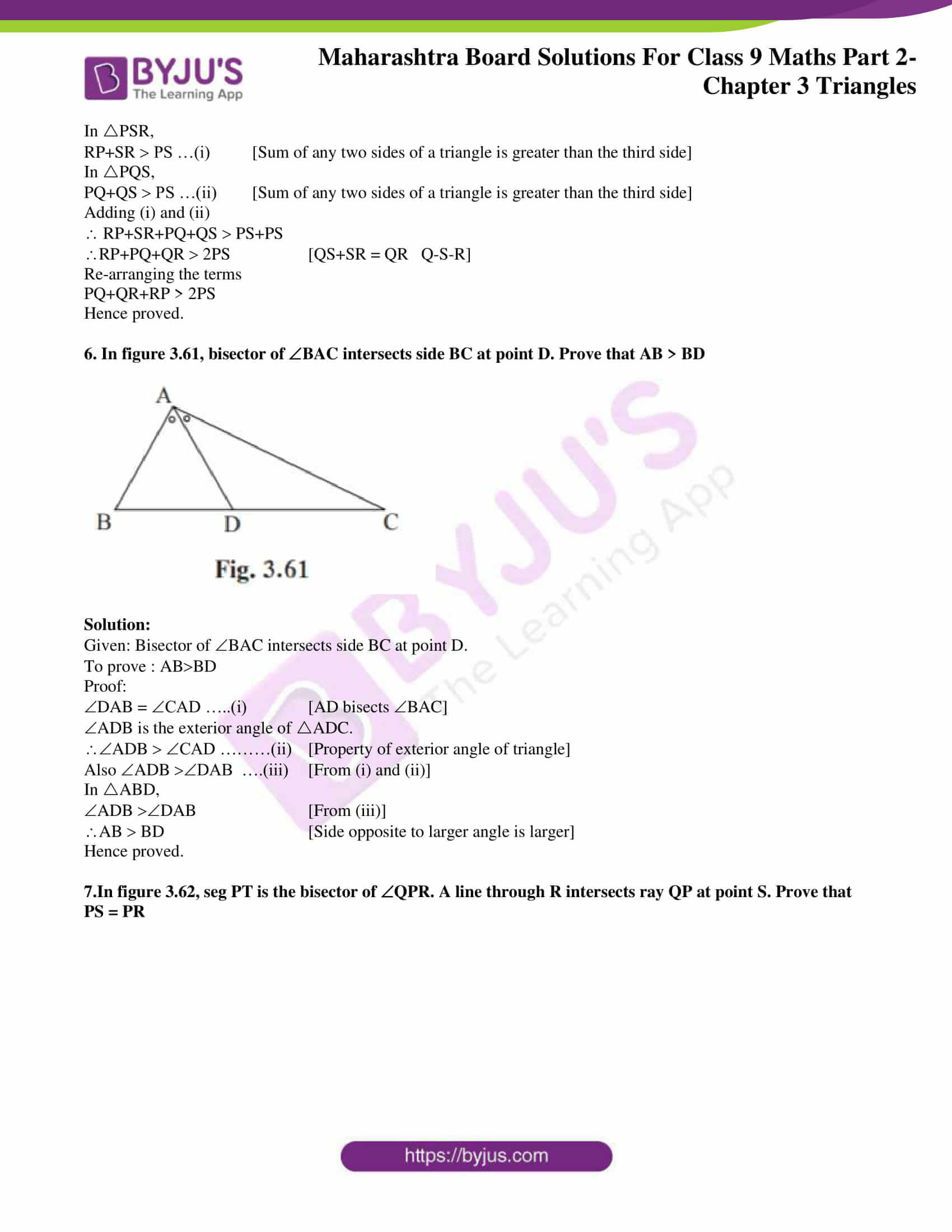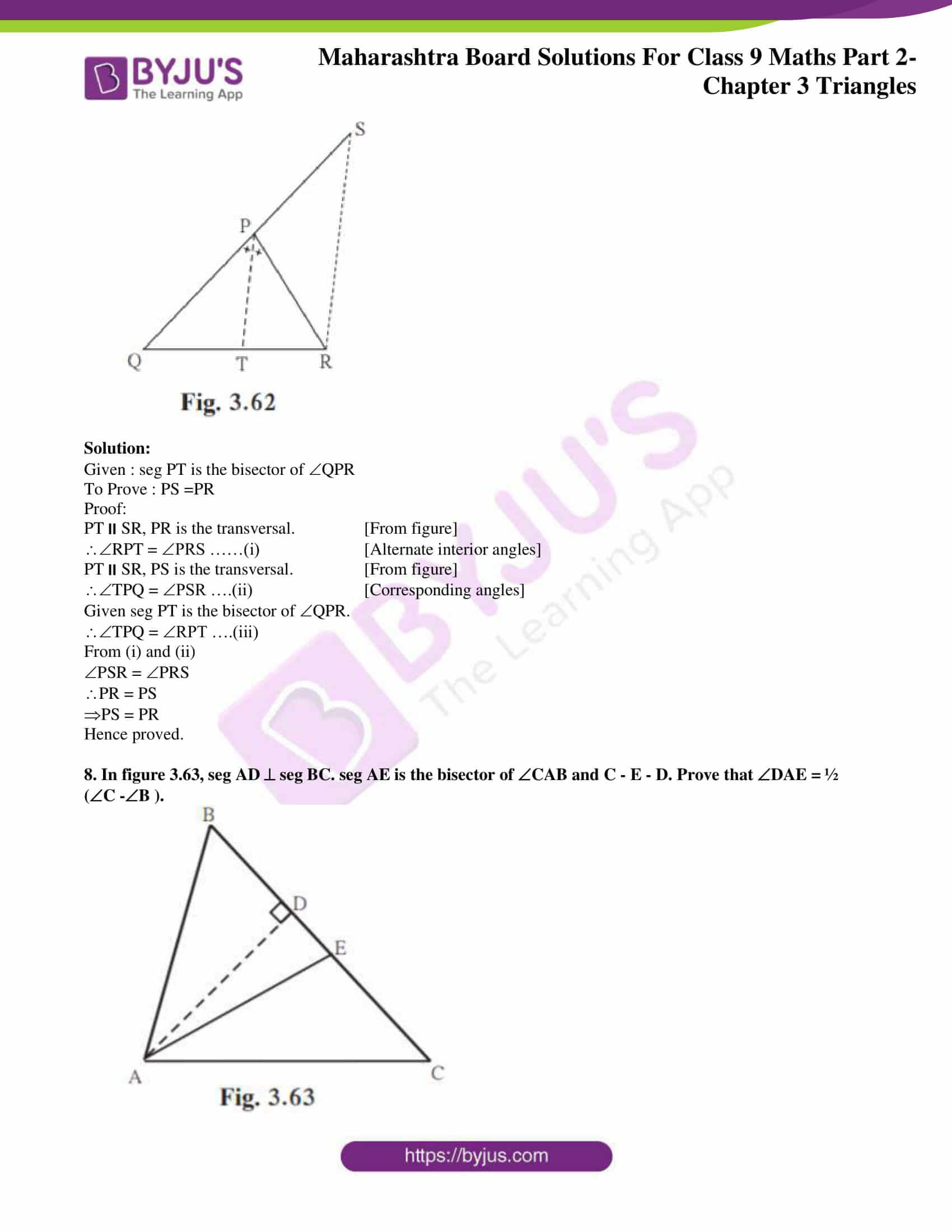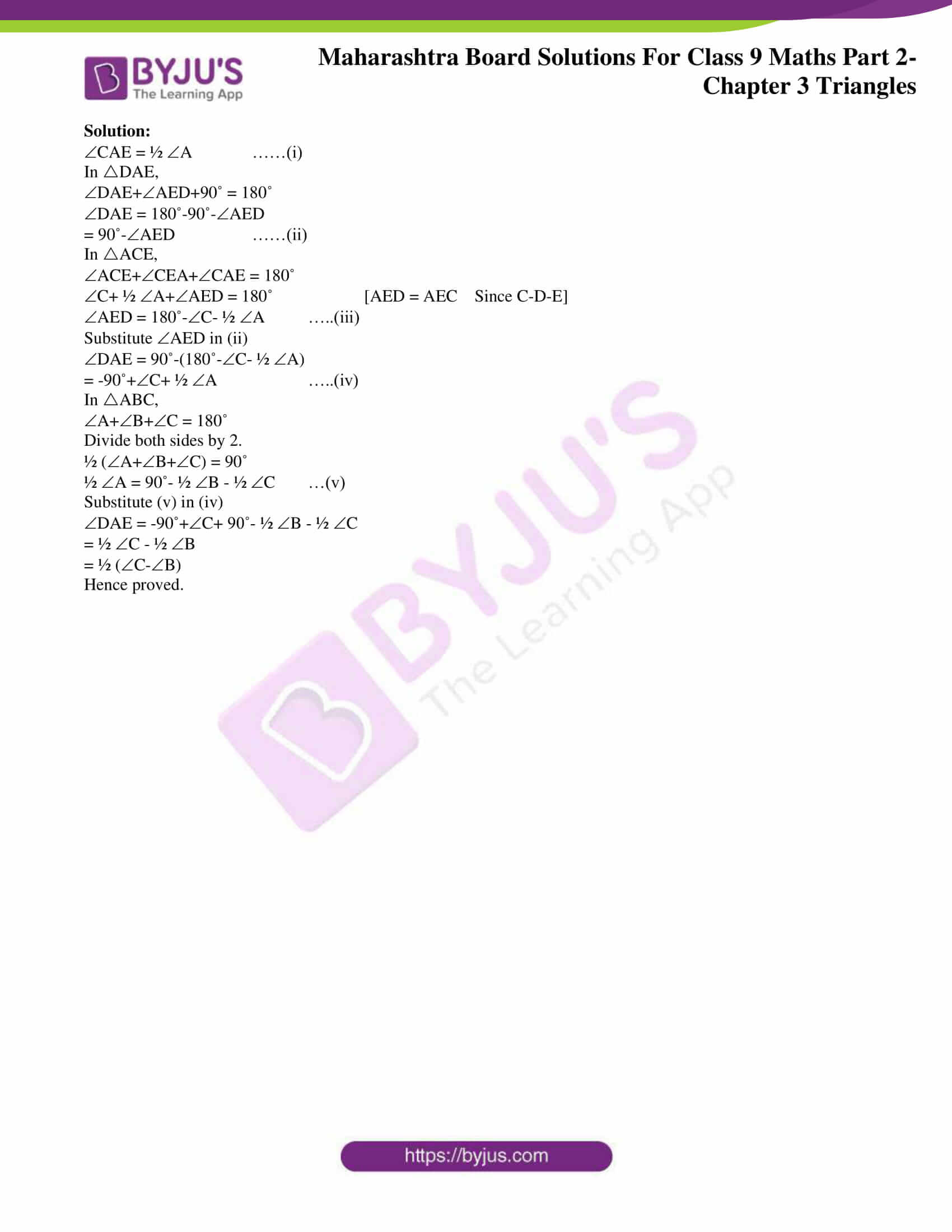### Access answers to Maths MSBSHSE Solutions For Class 9 Part 2 Chapter 3 – Triangles

Practice Set 3.1 Page 27

1. In figure 3.8, is an exterior angle of ABC. B = 40°, A = 70°. Find the measure of ACD.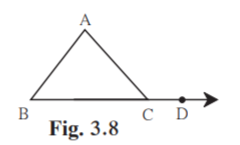Solution:

Given B = 40˚

A =70˚

ACD = A+B [Exterior angle]

ACD = 70+40 = 110˚.

Hence measure of ACD is 110˚.

2. In PQR, P = 70°, Q = 65° then find R.

Solution:

Given P = 70°

Q = 65°

P+Q+R = 180˚ [Angle sum property of triangle]

70+65+R = 180

R = 180-(70+65)

= 180-135 = 45˚

Hence measure of R is 45˚ .

3. The measures of angles of a triangle are x°, (x-20)°, (x-40)°. Find the measure of each angle.

Solution:

Given measures of angles of a triangle are x˚, (x-20)˚, (x-40)˚.

The sum of angles of a triangle is equal to 180˚.

x+x-20+x-40 = 180

3x-60 = 180

3x = 180+60 = 240

x = 240/3 = 80˚

x-20 = 80-20 = 60˚

x-40 = 80-40 = 40˚

Hence the measures of angles of a triangle are 80˚, 60˚ and 40˚.

4. The measure of one of the angles of a triangle is twice the measure of its smallest angle and the measure of the other is thrice the measure of the smallest angle. Find the measures of the three angles.

Solution:

Let the smallest angle be x.

Then measure of second angle = 2x [Given measure of one angle is twice the smallest angle]

Measure of third angle = 3x. [Given measure of other angle is thrice the smallest angle]

x+2x+3x = 180˚ [Angle sum property of a triangle]

6x = 180˚

x = 180/6 = 30˚

2x = 2×30 = 60˚

3x = 3×30 = 90˚

Hence the measures of the angles are 30˚, 60˚ and 90˚.

5. In figure 3.9, measures of some angles are given. Using the measures find the values of x, y, z.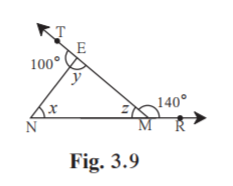Solution:

Given EMR = 140˚

z +EMR = 180˚ [Linear pair]

z +140˚ = 180˚

z = 180-140 = 40˚

Given TEN = 100˚

TEN +y = 180˚ [Linear pair]

100˚+y = 180˚

y = 180-100 = 80˚

x+y+z = 180˚ [Angle sum property of triangle]

x+80˚+40˚ = 180˚

x+120˚ = 180˚

x = 180˚-120˚ = 60˚

Hence the measures of the angles x, y and z are 60˚, 80˚ and 40˚ respectively.

6. In figure 3.10, line AB || line DE. Find the measures of DRE and ARE using given measures of some angles.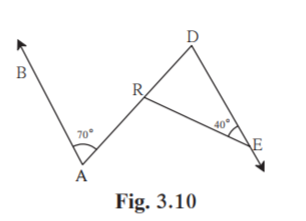Solution:

Given ABDE .

RDE = 70˚ [Alternate interior angles]

Given DER = 40˚

In RDE

RDE+DRE+DER = 180˚ [Angle Sum Property of triangle]

70˚+DRE+40˚ = 180˚

110˚+DRE = 180˚

DRE = 180-110 = 70˚

ARE+DRE = 180˚ [Linear pair]

ARE+70˚ = 180˚

ARE = 180-70 = 110˚

Hence measures of DRE and ARE are 70˚ and 110˚ respectively.

7. In ABC, bisectors of A and B intersect at point O. If C = 70°. Find measure of AOB.

Solution: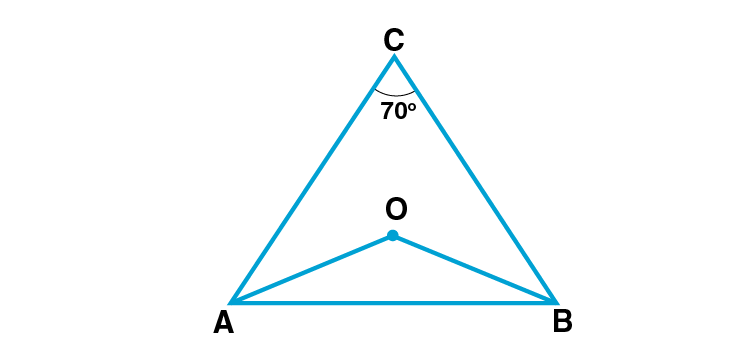Given C = 70˚

OAB = ½ CAB …….(i) [AO is the bisector of CAB]

OBA = ½ CBA …….(ii) [BO is the bisector of CBA]

In ABC

CAB+CBA+C = 180˚ [Angle sum property of triangle]

CAB+CBA+70˚ = 180˚

CAB+CBA = 180˚-70˚

CAB+CBA = 110

Multiply both sides by ½

½ CAB+½ CBA = 55˚

OAB+OBA = 55˚ ….(iii) [From (i) and (ii)]

In AOB

AOB+OAB+OBA = 180˚ [Angle sum property of triangle]

AOB+55˚ = 180˚ [From (iii)]

AOB = 180˚-55˚ = 125˚

Hence measure of AOB is 125˚.

8. In Figure 3.11, line AB || line CD and line PQ is the transversal. Ray PT and ray QT are bisectors of BPQ and PQD respectively. Prove that mPTQ = 90°.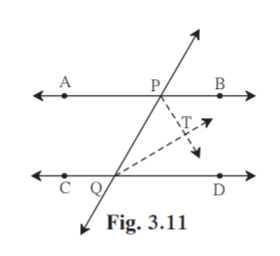Solution:

Given : ABCD . PQ is the transversal.

To prove: mPTQ = 90˚

Proof:

QPT = ½ BPQ …….(i) [PT is the bisector of BPQ]

PQT = ½ PQD …….(ii) [QT is the bisector of PQD]

Given ABCD . PQ is the transversal.

BPQ+PQD = 180˚ [Interior angles on same side of transversal are supplementary]

Multiply both side by ½ .

½ BPQ+ ½ PQD = 90˚

QPT+PQT = 90˚ ……(iii) [From (i) and (ii)]

In PTQ

QPT+PQT+PTQ = 180˚ [Angle sum property of triangle]

90˚+PTQ = 180˚ [From (iii)]

PTQ = 180˚-90˚

PTQ = 90˚

Hence proved.

9. Using the information in figure 3.12, find the measures of a, b and c.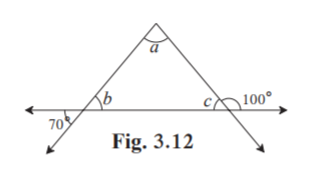Solution:

b = 70˚ [Vertically opposite angles]

c+100˚ = 180˚ [Linear pair]

c = 180-100 = 80˚

a+b+c = 180˚ [Angle sum property of triangle]

a+70˚+80˚ = 180˚

a +150˚ = 180˚

a = 180-150 = 30˚

Hence a = 30˚, b = 70˚ and c = 80˚.

10. In figure 3.13, line DE || line GF ray EG and ray FG are bisectors of DEF and DFM respectively. Prove that,

(i) DEG = ½ EDF

(ii) EF = FG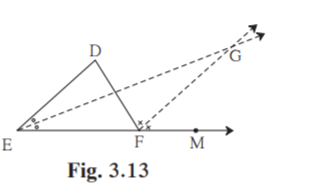Solution:

(i)Given DEGF

DEG = GEF = ½ DEF…….(i) [Ray EG bisects DEF]

DFG = GFM = ½ DFM……(ii) [Ray FG bisects DFM]

EDFG

DFG = EDF …..(iii) [Alternate interior angles]

In DEF

DFM = DEF+EDF [Exterior angle of a triangle is equal to the sum of remote interior angles]

2EDF = DEF+EDF [From (ii) and (iii)]

2EDF- EDF = DEF

EDF = DEF

EDF = 2DEG [From (i)]

DEG = ½ EDF

Hence proved.

(ii) Given DEGF.

EG is the transversal.

DEG = EGF ….(iv) [Alternate interior angles]

EGF = GEF [From (i) and (iv)]

EF = FG [Sides opposite to equal angles of a triangle are equal]

Hence proved.

Practice Set 3.2 Page 31

1. In each of the examples given below, a pair of triangles is shown. Equal parts of triangles in each pair are marked with the same signs. Observe the figures and state the test by which the triangles in each pair are congruent.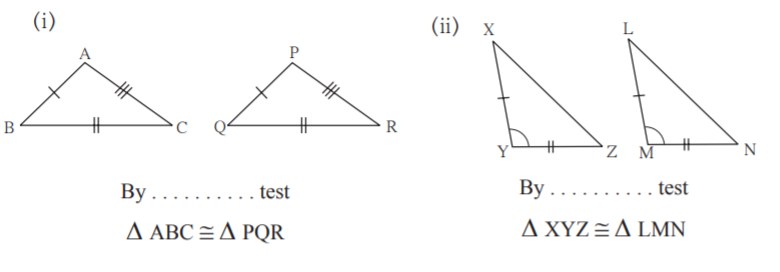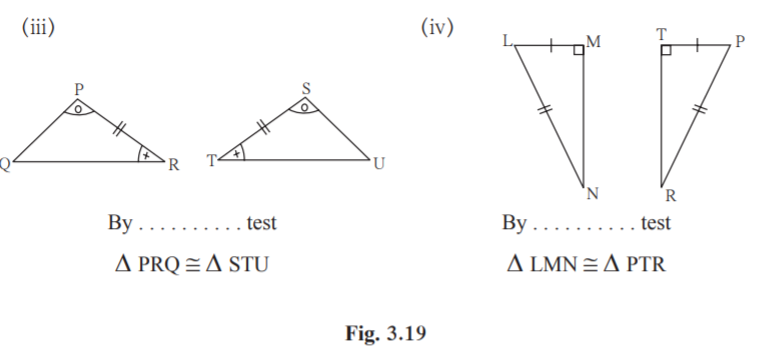Solution:

(i)From the figure , we know that the three sides of ABC are equal to the three sides of PQR.

By SSS test, ABC PQR

(ii)From the figure , we know that the two sides and the included angle of XYZ are equal to the two sides and the included angle of LMN.

By SAS test, XYZ LMN.

(iii) From the figure , we know that the two angles and the included side of PRQ are equal to the two angles and the included side of STU.

By ASA test, PRQ STU.

(iv) From the figure , we know that the right angles ,hypotenuse and a side of LMN are equal to the right angle, hypotenuse and a side of PTR.

By Hypotenuse side test, LMN PTR.

2. Observe the information shown in pairs of triangles given below. State the test by which the two triangles are congruent. Write the remaining congruent parts of the triangles.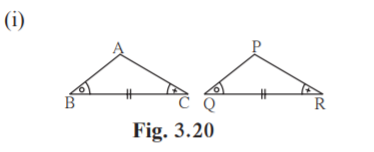From the information shown in the figure, in ABC and PQR

ABC PQR

seg BC seg QR

ACB PRQ

ABC PQR…… _____ test

BAC _____ corresponding angles of congruent triangles.

seg AB _____

and ______ seg PR {corresponding sides of congruent triangles}

Solution:

From the information shown in the figure, in ABC and PQR

ABC PQR

seg BC seg QR

ACB PRQ

ABC PQR…… ASA test

BAC QPR corresponding angles of congruent triangles.

seg AB seg PQ

and seg AC seg PR {corresponding sides of congruent triangles}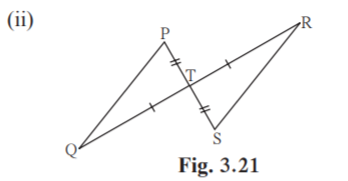From the information shown in the figure,,

In PTQ and STR

seg PT seg ST

PTQ STR….vertically opposite angles

seg TQ seg TR

PTQ STR…… _____ test

TPQ _____

and ____ TRS {corresponding angles of congruent triangles.}

seg PQ _____corresponding sides of congruent triangles.

Solution:

From the information shown in the figure,,

In PTQ and STR

seg PT seg ST

PTQ STR….vertically opposite angles

seg TQ seg TR

PTQ STR……. SAS test

TPQ TSR

and TQP TRS {corresponding angles of congruent triangles.}

seg PQ seg SR corresponding sides of congruent triangles.

3. From the information shown in the figure, state the test assuring the congruence of ABC and PQR. Write the remaining congruent parts of the triangles.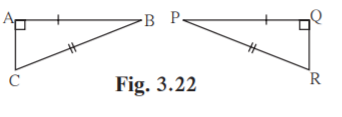Solution:

From given figure,

seg AB seg QP

seg BC seg PR

A = Q = 90˚

By Hypotenuse side test, ABC QPR

seg AC seg QR [c.s.c.t]

C = R [c.a.c.t]

B = P [c.a.c.t]

4. As shown in the following figure, in LMN and PNM, LM = PN, LN = PM. Write the test which assures the congruence of the two triangles. Write their remaining congruent parts.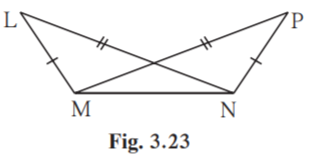Solution:

Given LM = PN

LN = PM

MN NM [Common side]

By SSS test LMN PNM

LMN PNM [c.a.c.t]

MNL NMP [c.a.c.t]

NLM MPN [c.a.c.t]

5. In figure 3.24, seg AB seg CB and seg AD seg CD. Prove that ABD CBD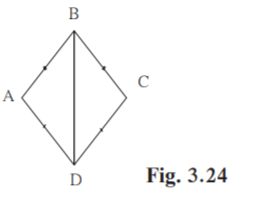Solution:

Proof:

seg AB seg CB [Given]

seg BD seg BD [Common side]

By SSS test, ABD CBD

Hence proved.

6. In figure 3.25, P R, seg PQ seg RQ Prove that, PQT RQS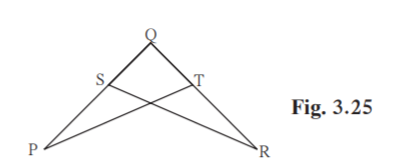Solution:

P R [Given]

seg PQ seg RQ [Given]

Q = Q [common angle]

By ASA test, PQT RQS

Hence proved.

Practice Set 3.3 Page 38

1. Find the values of x and y using the information shown in figure 3.37. Find the measure of ABD and ACD.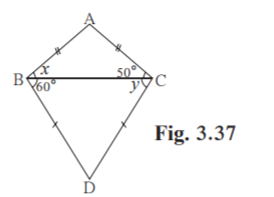Solution:

In ABC

seg AB seg AC [Given]

ACB = 50˚ [Given]

x = ACB = 50˚ [Isoceles angle theorem]

In BCD

seg BD seg CD [Given]

CBD = 60˚ [Given]

y = CBD = 60˚ [Isoceles angle theorem]

Hence measure of x and y are 50˚ and 60˚ respectively.

ABD = x+CBD [Angle addition property]

ABD = 50˚+60˚ = 110˚

Hence measure of ABD is 110˚.

ACD = ACB+y [Angle addition property]

ACD = 50˚+60˚ = 110˚

Hence measure of ACD is 110˚.

2. The length of hypotenuse of a right angled triangle is 15. Find the length of median of its hypotenuse.

Solution:

In a right angled triangle, the length of the median on its hypotenuse is half the length of the hypotenuse.

Given length of hypotenuse = 15

Length of median on its hypotenuse = 15/2 = 7.5

Hence the length of median on its hypotenuse is 7.5 units.

3. In PQR, Q = 90°, PQ = 12, QR = 5 and QS is a median. Find l(QS).

Solution: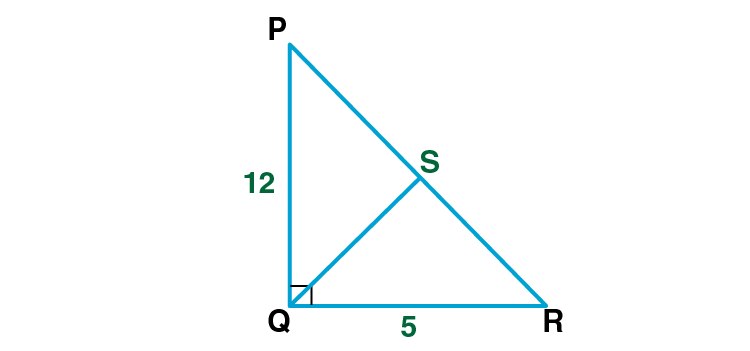In PQR

Given PQ = 12

QR = 5

Q = 90˚

PR2 = PQ2+QR2 [Pythagoras theorem]

PR2 = 122+52

PR2 = 144+25 = 169

Taking square root

PR = √169 = 13

In a right angled triangle, the length of the median on its hypotenuse is half the length of the hypotenuse.

QS = ½ PR

= ½ ×13 = 6.5

l(QS) is 6.5 units.

4. In figure 3.38, point G is the point of concurrence of the medians of PQR . If GT = 2.5, find the lengths of PG and PT.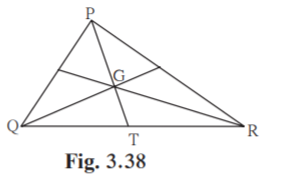Solution:

Given G is the point of concurrence of the medians of PQR.

The point of concurrence of medians of a triangle divides each median in the ratio 2 : 1.

PG:GT = 2:1

PG/GT = 2/1

PG/2.5 = 2/1 [Given GT = 2.5]

PG = 2.5×2 = 5

PT = PG+GT [P-G-T]

PT = 5+2.5 = 7.5

Hence length of PG and PT are 5 and 7.5 units respectively.

Practice Set 3.4 Page 43

1. In figure 3.48, point A is on the bisector of XYZ. If AX = 2 cm then find AZ.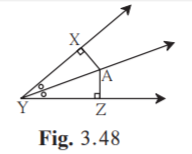Solution:

Given AX = 2 cm

Point A is on the bisector of XYZ.

Every point on the bisector of an angle is equidistant from the sides of the angle.

AZ = AX = 2cm.

Hence length of AZ is 2cm.

2. In figure 3.49, RST = 56°, seg PT ray ST, seg PR ray SR and seg PR seg PT Find the measure of RSP. State the reason for your answer.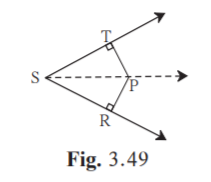Solution:

Given seg PT ray ST

seg PR ray SR

seg PR seg PT

Any point equidistant from sides of an angle is on the bisector of the angle.

P is a point on the bisector of TSR.

RST = 56˚ [Given]

RSP = ½ RST [Bisector of an angle divides it into two equal angles]

RSP = ½ ×56˚ = 28˚

Hence measure of RSP is 28˚.

3. In PQR, PQ = 10 cm, QR = 12 cm, PR = 8 cm. Find out the greatest and the smallest angle of the triangle.

Solution: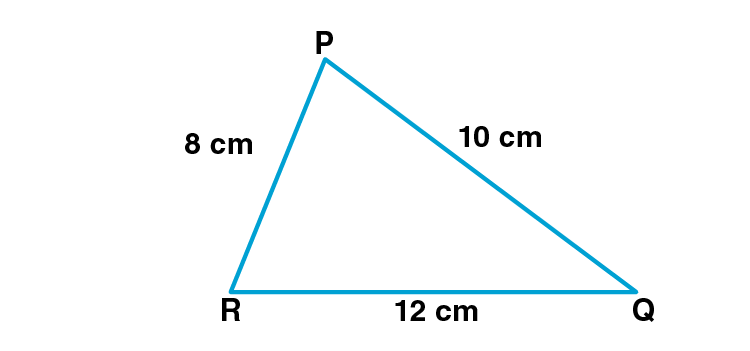Given PQ = 10 cm, QR = 12 cm, PR = 8 cm.

Here QR is the longest side and PR is the shortest side.

The angle opposite to the longest side is the largest angle and the angle opposite to the smallest side is the smallest angle .

RPQ is the largest angle and PQR is the smallest angle.

4. In FAN, F = 80°, A = 40° . Find out the greatest and the smallest side of the triangle. State the reason.

Solution: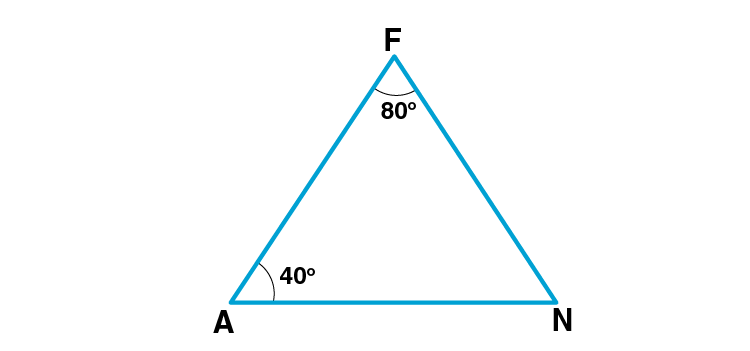Given F = 80°

A = 40°

F+A+N = 180˚ [Angle sum property of triangle]

80˚+40˚+N = 180˚

120˚+N = 180˚

N = 180˚-120˚ = 60˚

The side opposite to the largest angle is the largest side and the side opposite to the smallest angle is the smallest side.

Since 80˚60˚40˚

FNA

AN is the largest side and FN is the smallest side.

5. Prove that an equilateral triangle is equiangular.

Solution: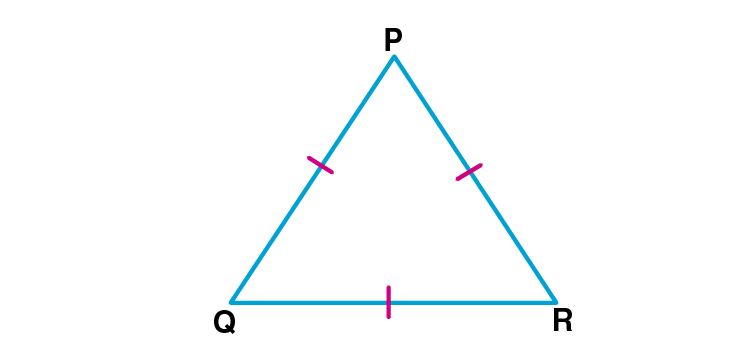Given: PQR is an equilateral triangle.

To prove : PQR is equiangular.

Proof:

seg PQ = seg PR ….(i) [Sides of equilateral triangle]

R = Q…..(i) [Isoceles triangle theorem]

seg PQ = seg QR [Sides of equilateral triangle]

R = P…….(ii) [Isoceles triangle theorem]

R = Q = P [From (i) and (ii)]

Hence PQR is equiangular.

6. Prove that, if the bisector of BAC of ABC is perpendicular to side BC, then ABC is an isosceles triangle.

Solution: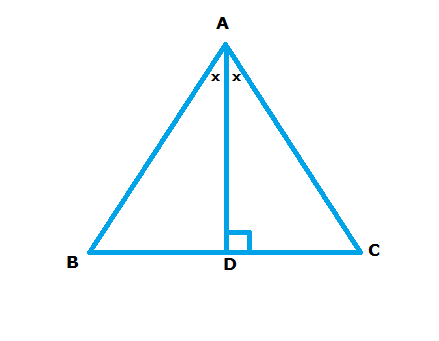AB AC [c.s.c.t]

ABC is an isosceles triangle.

Hence proved.

7. In figure 3.50, if seg PR seg PQ, show that seg PS > seg PQ.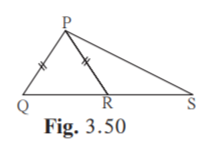Solution:

Given: seg PR seg PQ

To prove: seg PS > seg PQ

Proof:

seg PQ seg PR [Given]

PQR PRQ ….(i) [Isoceles triangle theorem]

PRQ is the exterior angle of PRS.

PRQ PSR….(ii) [Property of Exterior angle of a triangle]

PQR PSR [From (i) and (ii)]

Q S ….(iii)

In PQS,

Q S

seg PS seg PQ [The side opposite to the largest angle is the largest side]

Hence proved.

8. In figure 3.51, in ABC, seg AD and seg BE are altitudes and AE = BD. Prove that seg AD seg BE.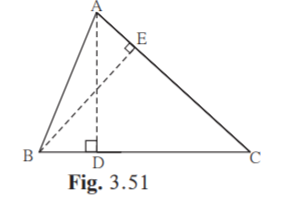Solution:

Proof:

In BAE and ABD ,

seg AE seg BD [Given]

seg BA seg AB [Common side]

BAE ABD [By Hypotenuse side test]

Hence proved.

Practice Set 3.5 Page 47

1. If XYZ ~ LMN, write the corresponding angles of the two triangles and also write the ratios of corresponding sides.

Solution:

Given XYZ ~LMN

Corresponding angles are X L

Y M

Z N

Ratio of corresponding sides = XY/LM = YZ/MN = XZ/LN

2. In XYZ, XY = 4 cm, YZ = 6 cm, XZ = 5 cm, If XYZ ~ PQR and PQ = 8 cm then find the lengths of remaining sides of PQR.

Solution:

Given XYZ ~ PQR

If two triangles are similar then their corresponding sides are in proportion and corresponding angles are congruent.

XY/PQ = YZ/QR = XZ/PR [Corresponding sides of similar triangles]

4/8 = 6/QR = 5/PR…..(i)

4/8 = 6/QR

4×QR = 8×6

QR = 8×6/4 = 48/4 = 12cm

Also, 4/8= 5/PR [From (i)]

4× PR = 8×5

PR = 8×5/4 = 40/4 = 10cm

Hence measure of QR and PR are 12cm and 10cm respectively.

3. Draw a sketch of a pair of similar triangles. Label them. Show their corresponding angles by the same signs. Show the lengths of corresponding sides by numbers in proportion.

Solution:

Two similar triangles are shown below.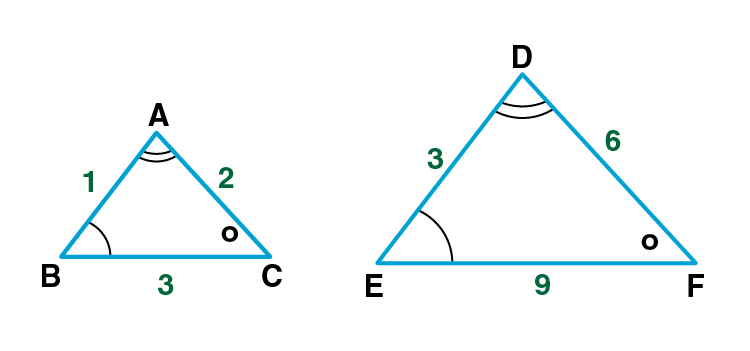ABC ~ DEF

Problem Set 3 Page 49

1. Choose the correct alternative answer for the following questions.

(i) If two sides of a triangle are 5 cm and 1.5 cm, the length of its third side cannot be . . . . . . . .

(A) 3.7 cm (B) 4.1 cm (C) 3.8 cm (D) 3.4 cm

Solution:

The sum of any two sides of a triangle is greater than the third side.

Here 1.5 + 3.4 = 4.95.

3.4cm cannot be length of third side.

Hence Option D is the answer.

(ii) In PQR, If R > Q then . . . . . . . . .

(A) QR > PR (B) PQ > PR (C) PQ < PR (D) QR < PR

Solution:

The side opposite to the largest angle is the largest side.

If R Q, then PQPR.

Hence Option B is the answer.

(iii) In TPQ, T = 65°, P = 95° which of the following is a true statement ?

(A) PQ < TP (B) PQ < TQ (C) TQ < TP < PQ (D) PQ < TP < TQ

Solution:

The angle opposite to the largest side is the largest angle.

PQ < TQ

Hence Option B is the answer.

2. ABC is isosceles in which AB = AC. Seg BD and seg CE are medians. Show that BD = CE.

Solution: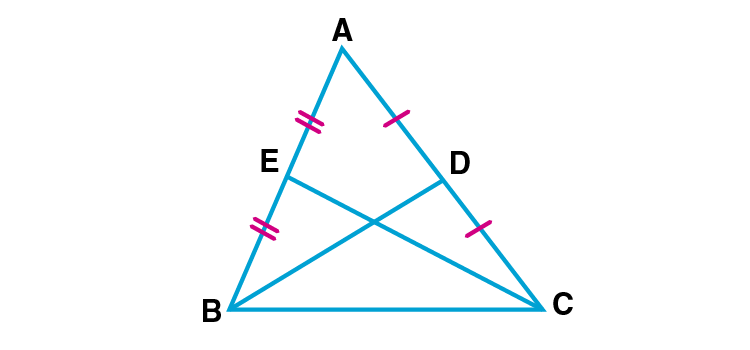Given: ABC is an isosceles triangle.

AB = AC

BD and CE are the medians.

To Prove: BD = CE

Proof:

AD = ½ AC …..(i) [D is the midpoint of AC]

AE = ½ AB …..(ii) [E is the midpoint of AB]

Given AB = AC….(iii)

AE = AD…. (iv) [From (i), (ii) and (iii)]

In ABD and ACE

seg AB = seg AC [Given]

seg AE = seg AD [From (iv)]

By SAS test ABD ACE.

BD = CE [c.s.c.t]

Hence proved.

3. In PQR, If PQ > PR and bisectors of Q and R intersect at S. Show that SQ > SR.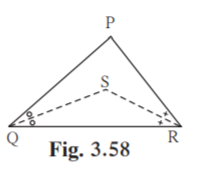Solution:

Given: In PQR, PQ > PR and bisectors of Q and R intersect at S.

To prove: SQ > SR

Proof:

SQR = ½ PQR ….(i) [QS is the bisector of Q]

SRQ = ½ PRQ ….(ii) [RS is the bisector of R]

In PQR,

PQ > PR [Given]

R > Q [Angle opposite to larger side is larger.]

Multiply both sides by ½

½ (R) > ½ (Q)

SRQ > SQR ….(iii) [From (i) and (ii)]

In SQR, from (iii)

SQ > SR [Side opposite to larger angle is larger]

Hence proved.

4. In figure 3.59, point D and E are on side BC of ABC, such that BD = CE and AD = AE. Show that ABD ACE.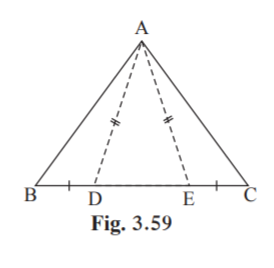Solution:

Given : Point D and E are on side BC of ABC

BD = CE

To Prove: ABD ACE

Proof:

Given seg AD = seg AE

ADE = AED ….(i) [Isoceles triangle theorem]

AED+AEC = 180˚….(iii) [Linear Pair]

Equate (ii) and (iii)

Substitute (i) in (iv)

In ABD and ACE,

seg BD seg CE [Given]

By SAS test, ABD ACE.

Hence proved.

5. In figure 3.60, point S is any point on side QR of PQR Prove that : PQ + QR + RP > 2PS.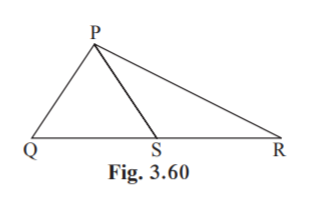Solution:

In PSR,

RP+SR PS …(i) [Sum of any two sides of a triangle is greater than the third side]

In PQS,

PQ+QS PS …(ii) [Sum of any two sides of a triangle is greater than the third side]

RP+SR+PQ+QS PS+PS

RP+PQ+QR 2PS [QS+SR = QR Q-S-R]

Re-arranging the terms

PQ+QR+RP > 2PS

Hence proved.

6. In figure 3.61, bisector of BAC intersects side BC at point D. Prove that AB > BD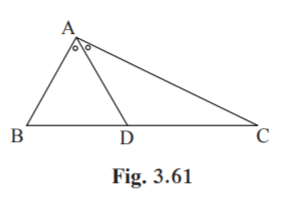Solution:

Given: Bisector of BAC intersects side BC at point D.

To prove : ABBD

Proof:

Also ADB DAB ….(iii) [From (i) and (ii)]

In ABD,

AB BD [Side opposite to larger angle is larger]

Hence proved.

7.In figure 3.62, seg PT is the bisector of QPR. A line through R intersects ray QP at point S. Prove that PS = PR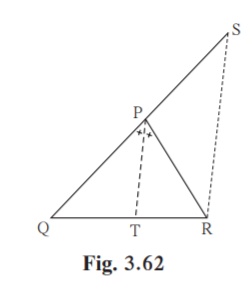Solution:

Given : seg PT is the bisector of QPR

To Prove : PS =PR

Proof:

PTSR, PR is the transversal. [From figure]

RPT = PRS ……(i) [Alternate interior angles]

PTSR, PS is the transversal. [From figure]

TPQ = PSR ….(ii) [Corresponding angles]

Given seg PT is the bisector of QPR.

TPQ = RPT ….(iii)

From (i) and (ii)

PSR = PRS

PR = PS

PS = PR

Hence proved.

8. In figure 3.63, seg AD seg BC. seg AE is the bisector of CAB and C – E – D. Prove that DAE = ½ (C -B ).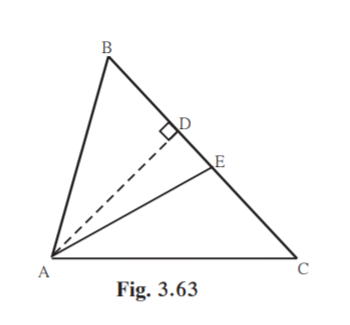Solution:

CAE = ½ A ……(i)

In DAE,

DAE+AED+90˚ = 180˚

DAE = 180˚-90˚-AED

= 90˚-AED ……(ii)

In ACE,

ACE+CEA+CAE = 180˚

C+ ½ A+AED = 180˚ [AED = AEC Since C-D-E]

AED = 180˚-C- ½ A …..(iii)

Substitute AED in (ii)

DAE = 90˚-(180˚-C- ½ A)

= -90˚+C+ ½ A …..(iv)

In ABC,

A+B+C = 180˚

Divide both sides by 2.

½ (A+B+C) = 90˚

½ A = 90˚- ½ B – ½ C …(v)

Substitute (v) in (iv)

DAE = -90˚+C+ 90˚- ½ B – ½ C

= ½ C – ½ B

= ½ (C-B)

Hence proved.

This chapter deals with the Angle bisector theorem and the Perpendicular bisector theorem. The concept of Median of a triangle and property of median on hypotenuse of a right angled triangle is explained in this chapter. Sign in to BYJU’S to get more information on the concepts of Triangles.

## Frequently Asked Questions on Maharashtra State Board Solutions for Class 9 Maths Part 2 Chapter 3 Triangles

### Are Maharashtra Board Class 9 Maths Part 2 Chapter 3 Solutions useful?

Yes, these solutions are very useful. Students are highly recommended to practise these solutions after revising the subjects, as they set the basis for the questions that could get asked often in the board exams. Students are encouraged to practise these questions at first. Then they can refer back to the solutions to analyse their performance. This will also help them to rectify the mistakes, so that they can avoid making any during the board exams.

### What are some of the concepts covered in Maharashtra Board Class 9 Maths Part 2 Chapter 3 solutions?

Maharashtra Board Class 9 Maths Part 2 Chapter 3 solutions deals with triangles. We also come across Theorem of remote interior angles, Congruence of triangles and Theorem of isosceles triangle in this chapter.

### Can we download these Maharashtra Board Class 9 Maths Part 2 Chapter 3 Triangles solutions?

Yes, Maharashtra Board Class 9  Maths Part 2 Chapter 3 solutions can be downloaded from the link given. We also provide the Scrollable PDF on the page and the questions and answers online on the webpage.